• 近年来，随着科学技术的不断发展，信息技术的日新月异，战争的智能化、信息化和一体化，使得任务规划成为高技术战争的重要支撑。自 1917年美国研制出第一架无人机以来，无人机先后经历了靶机、侦察机和诱饵机几个...
1 简介
近年来，随着科学技术的不断发展，信息技术的日新月异，战争的智能化、信息化和一体化，使得任务规划成为高技术战争的重要支撑。自 1917年美国研制出第一架无人机以来，无人机先后经历了靶机、侦察机和诱饵机几个发展阶段。无人机作为一种可重复使用的飞行器，以其结构简单、续航时间长、造价低、隐蔽性强和安全性高等优势，广泛地应用在信息化战争中执行监视、侦察、攻击、战场评估、精确打击、充当诱饵等任务，极大地提高了部队的指挥控制和多兵种协作作战的能力。早在越南战争、中东战争、科索沃战争、海湾战争及阿富汗战争中，无人机以其出色的表现受到世界各国的广泛关注。美国是无人机任务规划起步最早、发展最快、技术最先进的国家，在国外无人机技术发展的同时，中国也先后开启了无人机任务规划的研究。其中，北京航空航天大学、南京航空航天大学、西北工业大学和哈尔滨工业大学等高校先后成立了无人机相关研究机构，并取得了可喜的研究成果。自 2000 年以来一些民用无人机投入研制，无人机任务规划系统也从最初的单平台向多平台交互发展，目前有国防科技大学的多无人机协同任务资源分配与编队轨迹优化研究，哈尔滨工业大学的多无人机系统的协同目标分配和航迹规划方法研究、西北工业大学的无人机航路规划方法研究和北京航空航天大学的无人机路径规划技术研究等。此外，2015 年我国在纪念抗战 70 周年阅兵式上，首次展示了作战无人机，这表明了我国无人机的发展已经走向高新技术的前沿。随着全球格局的不断演变、军事科技的飞速发展，武器装备由机械化发展为信息化，战争方式较以前有了翻天覆地的变化。单无人机执行任务的局限性，使得多机协同作战成为当下研究的热点。多机协同执行高危任务，一方面可有效地降低毁伤概率，另一方面可提高战斗力。在多机协同执行任务中，当整个无人机系统达到最优时，并不能保证每架无人机均能达到最优。
指出了飞行器航迹规划与路径规划的区别;提出了一种给定威胁分布下的无人机路径规划算法.根据威胁分布情况构造无人机可能飞行的航路集,用voronoi图表示出来,采用Dijkstra算法搜索威胁分布图,求解粗略最短路径.在粗略最短路径的基础上,应用三次样条曲线和序列二次规划的方法求解最优路径.用Matlab进行仿真验证,证明了算法的有效性.
2 部分代码
%籍荤 切困 矫鼓饭捞磐涝聪促.

clear;
clc;

[Z, ref] = dted('n37.dt0');

N_s = 100;
x_s = linspace(1, N_s,N_s);
y_s = linspace(1, N_s,N_s);

x_2d_s = repmat(x_s,N_s,1);
y_2d_s = repmat(y_s',1,N_s);

x_1d_s = zeros(1,N_s*N_s);
y_1d_s = zeros(1,N_s*N_s);

z_1d_s = zeros(1,N_s*N_s);
z_2d_s = zeros(N_s,N_s);

cnt = 1;
for i = 1: N_s %1磊肺 父靛绰何盒
for j = 1: N_s
x_1d_s(cnt) = x_2d_s(i,j);
y_1d_s(cnt) = y_2d_s(i,j);
z_1d_s(cnt) = Z(i,j);
z_2d_s(i,j) = Z(i,j);
cnt = cnt+1;
end
end

%1窜拌 殿绊急瘤档 裙垫
% figure(1);
% set(gcf,'numbertitle','off','name', '殿绊急瘤档');
% contour(x_2d_s,y_2d_s,z_2d_s,'ShowText','on');
% xlabel('km');
% ylabel('km');

%% height
%2窜拌 困蛆钎扁

%%%%%%%%%%%%%%%%%%%%%%%%%%%%%%%%%%%%%%%%%%%%%%%%%%%%%%%%%%%%%%%%%%%%%%%%%%%%%%%%%%%%%%%%%%%%%%%%%%%%%%%%%%%%%%%%%%%%
% 厚青 臭捞(扁霖 臭捞)
flight_height = 700;% 厚青 臭捞焊促 角力 臭捞啊 臭酒 款亲捞 阂啊瓷茄 镑(厘局拱)
% 厘局拱狼 困摹绰 xn_new, yn_new, z_new 俊 历厘登绢乐澜
i_site = find(z_1d_s > flight_height);  %臭捞 蔼林绰何盒 角力肺 荤侩瞪 农扁 魄窜
xn_new = zeros(1,length(i_site));
yn_new = zeros(1,length(i_site));
z_new = zeros(1,length(i_site));

for sitetmp = 1:length(i_site) % 臭捞俊 狼秦辑 吧矾柳 xy困摹 历厘
xn_new(sitetmp) = x_1d_s(i_site(sitetmp));
yn_new(sitetmp) = y_1d_s(i_site(sitetmp));
z_new(sitetmp) = z_1d_s(i_site(sitetmp));
end

[vx, vy] = voronoi(xn_new,yn_new);

% 1凯狼 单捞磐 裙垫
vx1 = vx(1,:);
vy1 = vy(1,:);

% 2凯狼 单捞磐 裙垫
vx2 = vx(2,:);
vy2 = vy(2,:);

c_r = 1;
vx_new = vx;
vy_new = vy;

for i = 1 : length(xn_new)
% 1凯狼 单捞磐 裙垫
vx1 = vx_new(1,:);
vy1 = vy_new(1,:);

% 2凯狼 单捞磐 裙垫
vx2 = vx_new(2,:);
vy2 = vy_new(2,:);

r_sq = (vx1-xn_new(i)).^2+(vy1-yn_new(i)).^2;
idx1 = find((r_sq < c_r^2));

r_sq = (vx2-xn_new(i)).^2+(vy2-yn_new(i)).^2;
idx2 = find((r_sq < c_r^2));

idx = union(idx1,idx2);

vx_new(:,idx) = [];
vy_new(:,idx) = [];

end

% figure(2);
% set(gcf,'numbertitle','off','name', '困蛆钎扁');
% contour(x_2d_s,y_2d_s,z_2d_s,'ShowText','on');
% xlabel('km');
% ylabel('km');
% hold on;
% %plot(vx_new,vy_new,'b.-')
% plot(xn_new,yn_new,'r.');
% axis([0 N_s 0 N_s]);

%%
%3窜拌 款亲啊瓷 版肺 钎扁

% figure(3);
% set(gcf,'numbertitle','off','name', '版肺钎扁');
% contour(x_2d_s,y_2d_s,z_2d_s,'ShowText','on');
% xlabel('km');
% ylabel('km');
% hold on;
% plot(vx_new,vy_new,'b.-')
% plot(xn_new,yn_new,'r.');
% axis([0 N_s 0 N_s]);

%4窜拌 基荤捞飘 版肺 钎扁
th = 0:0.01:2*pi; %盔阑 弊府扁困茄 阿档 硅凯
%c_cx = 15; c_cy = 17; c_r =5;
c_cx = 0; c_cy = 0; c_r = 0;
xc = c_r*cos(th)+c_cx;
yc = c_r*sin(th)+c_cy;

vx1 = vx_new(1,:);
vy1 = vy_new(1,:);
r_sq = (vx1-c_cx).^2+(vy1-c_cy).^2;
idx1 = find((r_sq < c_r^2));

vx2 = vx_new(2,:);
vy2 = vy_new(2,:);

r_sq = (vx2-c_cx).^2+(vy2-c_cy).^2;
idx2 = find((r_sq < c_r^2));

idx = union(idx1,idx2);

lgd = legend('免惯痢','档馒痢');
lgd.AutoUpdate = 'off';
contour(x_2d_s,y_2d_s,z_2d_s,'ShowText','on');
xlabel('km');
ylabel('km');

% plot(vx_new,vy_new,'b.-');
plot(xn_new,yn_new,'r.');
h = fill(xc,yc,'red');
set(h,'facealpha',.5);
axis([0 N_s 0 N_s]);

% 急雀 版肺 醚 辨捞
path_length_all = 0;

part_length = [];

% 弥措 惑铰 臭捞(弥措 款侩 绊档)
max_flight_height = 1000;

%% 惑铰
%%%%%%%%%%%%%%%%%%%%%%%%%%%%%
% z绵 埃拜
z_alpha = 20;

% 厘局拱 困摹 颇厩
x_obs = [];
y_obs = [];

count_obs = 0;

% 流急狼 规沥侥 : y = ax + b
res = polyfit(xpoint, ypoint, 1);
x_equation = xpoint(1) : (x_s(2)-x_s(1)) : xpoint(2);
y_equation = res(1)*x_equation + res(2);

% 惑铰 棺 窍碍困茄 臭捞蔼 眠免 函荐
z_lift_height = [];
% flight height甫 馆康茄 臭捞 沥焊
lift_height = [];

y_index = [];

%%%%%%%%%%%%%%%%%%%%%%%%%%%%%%%%%%%%%%%%%%%%%%%%%%%%%%%%%%%%%%%%%%%%%%%%%%%%%%%%%
% 流急 版肺俊 乐绰 痢甸吝 老摹窍绰 y 瘤痢 茫扁
for i = 1 : length(y_equation)

flag = 0;

for j = 2 : N_s-1
num = find( y_equation(i) == y_s(j) );

if(num)
y_equation(i) = y_s(j);
flag = 1;
break;

else
flag = 0;

end

end

if(flag == 0)
y_equation(i) = y_s(knnsearch(y_s', y_equation(i)));

end

y_index(i) = knnsearch(y_s', y_equation(i));

end

%x_equation
%y_equation

lift_height = [];

obs_index = [];

% 厘局拱狼 俺荐
count_obs = 0;

% 流急 版肺俊 乐绰 痢甸吝 老摹窍绰 z 瘤痢 茫扁
for k = 1 : length(y_equation)
for i = 1 : N_s

% x客 悼老茄 瘤痢 2d俊辑 茫扁
if(find( x_equation(k) == x_s(i) ))
z_lift_height(k) = z_2d_s( (y_index(k)-1)*N_s + i );

if(z_lift_height(k) < flight_height)
lift_height(k) = flight_height;

%             elseif(z_lift_height(k) == flight_height)
count_obs = count_obs + 1;
%                 lift_height(k) = z_lift_height(k);
obs_index(count_obs) = k;

else
lift_height(k) = z_lift_height(k);

end

end

end

end

% z_lift_height

% plot3(x_equation, y_equation, lift_height + z_alpha, 'black', 'LineWidth',4);

%% 惑铰阿 备窍扁

% obs_index

% 厘局拱狼 困摹 沥焊 历厘
x_obs = [];
y_obs = [];

th = [];

% x_equation
% y_equation
% lift_height

% for k = 1 : length(y_equation)
%
%     if((lift_height(k)-3) == flight_height)
%         % 厘局拱 困摹 颇厩
% %         count_obs = count_obs + 1;
%         x_obs(count_obs) = x_equation(k);
%         y_obs(count_obs) = y_equation(k);
%
%         z_obs(count_obs) = lift_height(k);
%
%     end
%
% end

temp_away = 5;

% k == obs_index(i)
for i = 1 : count_obs

k = obs_index(i);

if( (k > temp_away) && (k ~= obs_index(end)) )

%         disp('yes');

if( lift_height(k) < lift_height(k+1) )
%             disp('up');

%         y_equation(k+1)
%         y_equation(k)
%         x_equation(k+1)
%         x_equation(k)

a = ( (lift_height(k+1) - lift_height(k-temp_away)) / (x_equation(k+1) - x_equation(k-temp_away)) );
th_temp = atan(a);
th(i) = th_temp * 180 / pi;

elseif( lift_height(k) < lift_height(k-1) )
%         disp('down');

else
%         disp('nothing');

end

end

end

% x_obs
% y_obs
% z_obs

count_obs

th

%% 傈眉 惑铰 版肺 辨捞 备窍扁

% 惑铰 棺 窍碍 版肺 醚 辨捞
lift_length = 0;
%z_lift_height
lift_length_part = [];
%lift_heigth

for i = 1 : length(y_equation)-1
lift_length_part(i) = sqrt( (x_equation(i+1)-x_equation(i))^2 + (y_equation(i+1)-y_equation(i))^2 + ( (lift_height(i+1)-lift_height(i))/1000 )^2 );

lift_length = lift_length + sqrt( (x_equation(i+1)-x_equation(i))^2 + (y_equation(i+1)-y_equation(i))^2 + ( (lift_height(i+1)-lift_height(i))/1000 )^2 );

end

disp('傈眉 惑铰 版肺 辨捞');
lift_length

%%
for mainloof = 1 : length(order)-1

xy_start = [xpoint(order(mainloof)) ypoint(order(mainloof))];
xy_dest = [xpoint(order(mainloof+1)) ypoint(order(mainloof+1))];

%惑铰 棺 窍碍
%%%%%%%%%%%%%%%%%%%%%%%%%%%%%%%%%%%%%%%%%%%%%%%%%%%%%%%%%%%%%%%%%%%%%%%%%%%%%%%
% 眠啊

x_obs_pos = [];
y_obs_pos = [];

x_obs_location = [];
y_obs_location = [];

obs_pos = [];

%%%%%%%%%%%%%%%%%%%%%%%%%%%%%
% 厘局拱 困摹 颇厩
x_obs = [];
y_obs = [];

count_obs = 0;

% 流急狼 规沥侥 : y = ax + b
res = polyfit(xpoint, ypoint, 1);
x_equation = xpoint(1) : (x_s(2)-x_s(1)) : xpoint(2);
y_equation = res(1)*x_equation + res(2);

% 惑铰 棺 窍碍困茄 臭捞蔼 眠免 函荐
z_lift_height = [];
% flight height甫 馆康茄 臭捞 沥焊
lift_height = [];

y_index = [];

%%%%%%%%%%%%%%%%%%%%%%%%%%%%%%%%%%%%%%%%%%%%%%%%%%%%%%%%%%%%%%%%%%%%%%%%%%%%%%%%%
% 流急 版肺俊 乐绰 痢甸吝 老摹窍绰 y 瘤痢 茫扁
for i = 1 : length(y_equation)

flag = 0;

for j = 2 : N_s-1
num = find( y_equation(i) == y_s(j) );

if(num)
y_equation(i) = y_s(j);
flag = 1;
break;

else
flag = 0;

end

end

if(flag == 0)
y_equation(i) = y_s(knnsearch(y_s', y_equation(i)));

end

y_index(i) = knnsearch(y_s', y_equation(i));

end

%x_equation
%y_equation

% 流急 版肺俊 乐绰 痢甸吝 老摹窍绰 z 瘤痢 茫扁
for k = 1 : length(y_equation)
for i = 1 : N_s

% x客 悼老茄 瘤痢 2d俊辑 茫扁
if(find( x_equation(k) == x_s(i) ))
z_lift_height(k) = z_2d_s( (y_index(k)-1)*N_s + i );

if(z_lift_height(k) < flight_height)
lift_heigth(k) = flight_height;

z_lift_height(k) = 300 + z_lift_height(k);

else
% 厘局拱 困摹 颇厩
count_obs = count_obs + 1;
x_obs(count_obs) = x_equation(k);
y_obs(count_obs) = y_equation(k);

lift_heigth(k) = z_lift_height(k);

end

end

end

end

%z_lift_height
lift_length_part = [];
%lift_heigth

%     for i = 1 : length(y_equation)-1
%         lift_length_part(i) = sqrt( (x_equation(i+1)-x_equation(i))^2 + (y_equation(i+1)-y_equation(i))^2 + ( (lift_heigth(i+1)-lift_heigth(i))/1000 )^2 );
%
%         lift_length = lift_length + sqrt( (x_equation(i+1)-x_equation(i))^2 + (y_equation(i+1)-y_equation(i))^2 + ( (lift_heigth(i+1)-lift_heigth(i))/1000 )^2 );
%
%     end

%%%%%%%%%%%%%%%%%%%%%%%%%%%%%%%%%%%%%%%%%%%%%%%%%%%%%%%%%%%%%%%%%%%%%%%%%%%%%%%%

z_alpha = 10;

%     if(z_lift_height < flight_height)
%         z_lift_height = z_lift_height + flight_height;
%
%     end

% 惑铰 棺 窍碍 角氰
figure(3)
% making 3D MAP
meshc(x_2d_s, y_2d_s, z_2d_s);
hold on;
%     plot3(x_equation, y_equation, z_lift_height + z_alpha, 'black', 'LineWidth',4);
plot3(x_equation, y_equation, lift_heigth + z_alpha, 'red', 'LineWidth',4);

for i = 1 : length(x_equation)

x_obs_pos = find(x_equation(i) == xn_new);
y_obs_pos = find(y_equation(i) == yn_new);

end

for i = 1 : length(x_obs_pos)
obs_pos = find(x_obs_pos(i) == y_obs_pos);
%obs_pos

if(obs_pos)
x_obs_location(i) = xn_new(x_obs_pos(obs_pos));
y_obs_location(i) = yn_new(y_obs_pos(obs_pos));

end

end

% 咯扁 鳖瘤
%%%%%%%%%%%%%%%%%%%%%%%%%%%%%%%%%%%%%%%%%%%%%%%%%%%%%%%%%%%%%%%%%%%%%%%%%%%%%%%%%%%

vx_all = vx_new(:);
vy_all = vy_new(:);

dr = kron(ones(length(vx_all),1),xy_start)-[vx_all vy_all];
[min_val,min_id] = min(sum(dr.^2,2));

vx_new = [vx_new [xy_start(1); vx_all(min_id)]];
vy_new = [vy_new [xy_start(2); vy_all(min_id)]];

dr = kron(ones(length(vx_all),1),xy_dest)-[vx_all vy_all];
[min_val,min_id] = min(sum(dr.^2,2));

vx_new = [vx_new [xy_dest(1); vx_all(min_id)]];
vy_new = [vy_new [xy_dest(2); vy_all(min_id)]];

xy_all = unique([vx_new(:) vy_new(:)],'rows');
dv = [vx_new(1,:); vy_new(1,:)] - [vx_new(2,:); vy_new(2,:)];
edge_dist = sqrt(sum(dv.^2));

G = sparse(size(xy_all,1),size(xy_all,1));

for kdx = 1:length(edge_dist)
xy_s = [vx_new(1,kdx) vy_new(1,kdx)];
idx = find(sum((xy_all-kron(ones(size(xy_all,1),1),xy_s)).^2,2)==0);
xy_d = [vx_new(2,kdx) vy_new(2,kdx)];
jdx = find(sum((xy_all-kron(ones(size(xy_all,1),1),xy_d)).^2,2)==0);
G(idx,jdx) = edge_dist(kdx);
G(jdx,idx) = edge_dist(kdx);
end

st_idx = find(sum((xy_all-kron(ones(size(xy_all,1),1),xy_start)).^2,2)==0);
dest_idx = find(sum((xy_all-kron(ones(size(xy_all,1),1),xy_dest)).^2,2)==0);

[dist,paths,pred] = graphshortestpath(G,st_idx,dest_idx);
xy_opt_path = xy_all(paths,:);
% for i = 1 : length(xy_opt_path)
%
% end
%1凯狼 单捞磐 裙垫
d_r = 1.4;
d_r2 = 0.9;
xd = xy_opt_path(:,1);
yd = xy_opt_path(:,2);
trues = zeros(1,length(xy_opt_path));
for i = 1 : length(xy_opt_path)
r_sq = (xd(i)-xn_new).^2+(yd(i)-yn_new).^2;
idx1 = find((r_sq < d_r^2));
r_sq = (xd(i)-xc).^2+(yd(i)-yc).^2;
idx2 = find((r_sq < d_r2^2));

if (isempty(idx1)==1) && (isempty(idx2)==1)
trues(i) = 1;
end
end

%     % 流急 版肺 单捞磐
%     ttemp_xy_path_all{mainloof} = xy_opt_path;
%
%     rootCount = 0;
%
%     % 傈眉 版肺 辨捞 备埃喊 拌魂
%     path1 = 0;
%
%     for i = 1 : length(xy_opt_path)-1
%
%         path_part = sqrt((xy_opt_path(i,1) - xy_opt_path(i+1,1))^2 + (xy_opt_path(i,2) - xy_opt_path(i+1,2))^2);
%         path1 = path_part + path1;
%         %part_length(i) = path_part;
%
%     end
%
%     path_length(mainloof) = path1;
%     %part_length_all{mainloof} = part_length;
%
% %         path_length_all = path_length(mainloof) + path_length_all;
%     path_length_all = path1 + path_length_all;

figure(1);
plot(xy_start(1),xy_start(2),'S',xy_dest(1),xy_dest(2),'D','MarkerSize',10, 'MarkerFaceColor','black');
%     plot(xy_opt_path(:,1),xy_opt_path(:,2), 'green', 'LineWidth',4);

%     for i = 1 : length(xy_opt_path)
%         if trues(i) == 0
%             xx = [xy_opt_path(i,1) xy_opt_path(i+1,1)];
%             yy = [xy_opt_path(i,2) xy_opt_path(i+1,2)];
%             plot(xx,yy, 'r-','LineWidth',3);
%         end
%     end
%唱吝俊 秦搬

figure(2)
% making 3D MAP
meshc(x_2d_s, y_2d_s, z_2d_s);
hold on;

%%%%%%%%%%%%%%%%%%%%%%%%%%%%%%%%%%%%%%%%%%%%%%%%%%%%%%%%%%%%%%%%%%%%%%%%%%%%%
% z 绵狼 单捞磐 档免

zd = [];
z_data = [];

for i = 1 : length(xd)

pos_x = 10000;
pos_y = 10000;

x_data = 0;
y_data = 0;

% 啊厘 啊鳖款 困摹肺 瘤沥
for j = 1 : length(x_s)

x_length = abs( x_s(j) - xd(i) );
y_length = abs( y_s(j) - yd(i) );

if( pos_x > x_length )
pos_x = x_length;

x_data = x_s(j);
else
pos_x = pos_x;
x_data = x_data;
end

if( pos_y > y_length )
pos_y = y_length;
y_data = y_s(j);
else
pos_y = pos_y;
y_data = y_data;
end

end

% 秦寸 困摹狼 x谅钎狼 蔼苞 y谅钎狼 蔼阑 茫酒 历厘
temp_pos_x = find( x_data == x_2d_s );
temp_pos_y = find( y_data == y_2d_s );
temp_pos_z = 0;

for k = 1 : length(temp_pos_x)
temp = find( temp_pos_x(k) == temp_pos_y );

if( temp )
temp_pos_z = temp;

z_data(i) = z_2d_s(temp_pos_z);

% 厚青绊档 力茄 拌魂
if( z_data(i) < flight_height )
z_data(i) = flight_height + height;
end

else
temp_pos_z = temp_pos_z;

end

end

end

%z_data

% 急雀 流急 版肺 单捞磐
ttemp_xy_path_all{mainloof} = xy_opt_path;

rootCount = 0;

% 傈眉 版肺 辨捞 备埃喊 拌魂
path1 = 0;

for i = 1 : length(xy_opt_path)-1

path_part = sqrt((xy_opt_path(i,1) - xy_opt_path(i+1,1))^2 + (xy_opt_path(i,2) - xy_opt_path(i+1,2))^2 + ( (z_data(i+1)-z_data(i)) /1000)^2);
path1 = path_part + path1;
part_length(i) = path_part;

end

path_length(mainloof) = path1;
part_length_all{mainloof} = part_length;

%     path_length_all = path_length(mainloof) + path_length_all;
path_length_all = path1 + path_length_all;

% using plot3 function   ex. plot3(x1, y1, z1, ...)
plot3(xy_opt_path(:,1),xy_opt_path(:,2), z_data, 'red', 'LineWidth',4);

%%%%%%%%%%%%%%%%%%%%%%%%%%%%%%%%%%%%%%%%%%%%%%%%%%%%%%%%%%%%%%%%%%%%%%%%%%%%%

end

disp('傈眉 急雀 版肺 辨捞');
path_length_all

3 仿真结果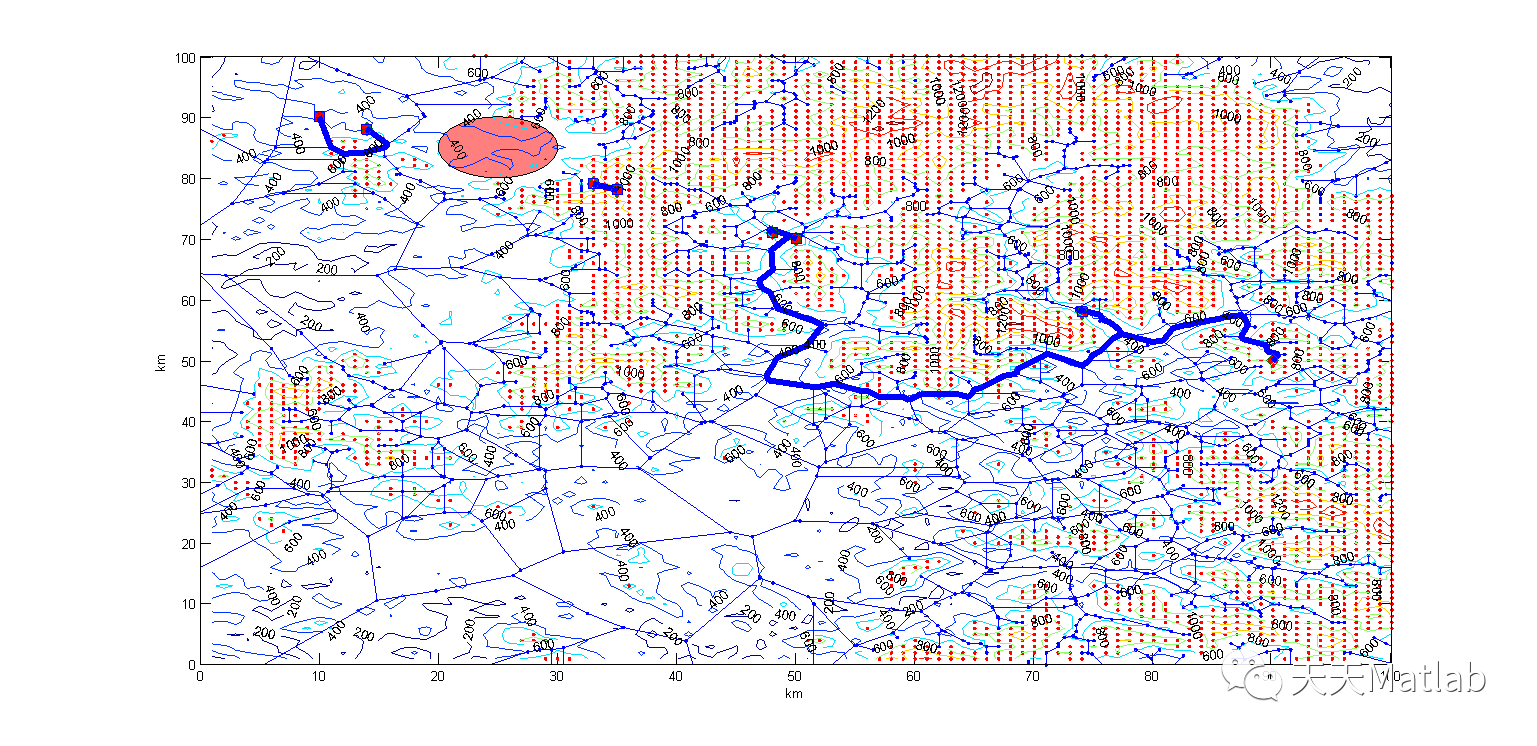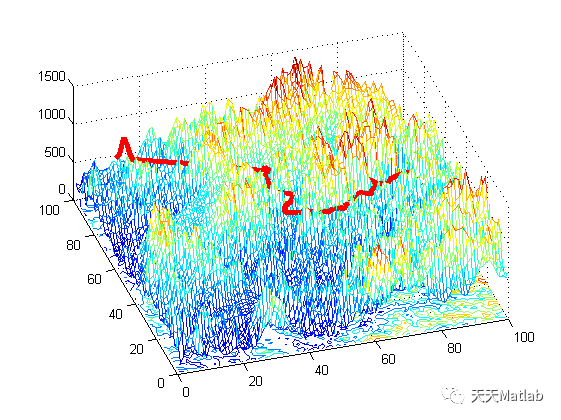4 参考文献
符小卫, and 高晓光. "一种无人机路径规划算法研究." 系统仿真学报 01(2004):20-21.​展开全文开发语言
• 路径规划】基于粒子群的三维无人机路径规划matlab源码
• 用于路径规划的7种算法源代码，包括A星，遗传算法GA,快速随机搜索树RRT,概率图PRM等，适合移动机器人的路径规划
• 路径规划】基于蚁群算法实现无人机路径规划matlab源码.zip简介
• 路径规划】基于粒子群的三维无人机路径规划matlab源码.zip简介
• 路径规划】基于粒子群的三维无人机路径规划matlab源码2.zip简介
• 若(i，j)为最差蚂蚁路径中的一条边，且不是最优蚂蚁路径中的边，则该路径的信息素按如下调整：\ 5.6 代码  %% 该函数用于演示基于蚁群算法的三维路径规划算法 %% 清空环境 clc clear %% 数据初始化 %下载数据 ...

5.1 介绍
蚁群优化(ACO)是群体智能的一部分，它模仿蚂蚁的合作行为来解决复杂的组合优化问题。它的概念是由Marco Dorigo和他的同事提出的，当他们观察到这些生物在寻找食物时所采用的相互交流和自我组织的合作方式时，他们感到很惊讶。他们提出了执行这些策略的想法，为不同领域的复杂优化问题提供了解决方案，并获得了广泛的欢迎[1, 2]。
蚁群算法是一组被称为人工蚂蚁的软件代理，它们为特定的优化问题寻找好的解决方案。蚁群算法是通过将问题映射成一个加权图来实现的，在加权图中，蚂蚁沿着边缘移动，寻找最佳路径。
蚁群研究(实际上是真正的蚂蚁)始于1959年，当时皮埃尔•保罗•格拉斯(Pierre Paul Grasse)发明了“协同”理论，解释了白蚁的筑巢行为。之后于1983年Deneubourg和他的同事们对蚂蚁的集体行为进行了研究。1988年，Mayson和Manderick发表了一篇关于蚂蚁的自组织行为的文章。最终在1989年，Goss, Aron, Deneubour, and Pasteelson在其研究工作(阿根廷蚂蚁的集体行为)中提出了蚁群算法的基本思想，同年，Ebling及其同事提出了一食物定位模型。1992年，Marco Dorigo(Dorigo, 1992)在其博士论文中提出了蚂蚁系统(Ant System)。一些研究人员将这些算法扩展到各个研究领域的应用中，Appleby和英国电信主管发表了第一个在电信网络中的应用，后来Schoonderwoerd和他的同事在1997年对其进行了改进。在2002年，它被应用于贝叶斯网络中的调度问题。
蚁群算法的设计是基于蚂蚁搜索巢穴和食物位置之间短路径的能力，这可能会因蚂蚁的种类而有所不同。近年来，研究人员对蚁群算法的应用结果进行了研究，结果表明，所使用的大多数人工蚂蚁并不能提供最好的解决方案，而精英蚁群通过重复的交换技术提供了最好的解决方案。他们进一步指出，如果考虑相同的参数，混合和交换方法的性能优于蚁群系统(ACS)。
基于这一理论，研究人员提出了一种专门的蚁群优化方法，可以模拟特定种类的蚂蚁，如黑蚁(Lasius niger或Irodomyrex humilis)，著名的阿根廷蚂蚁。
5.2 人工蚂蚁的概念
自然生物的合作行为常常被证明是解决现实生活中各种复杂问题的一个很好的方法。生物的协作行为吸引了研究人员将它们的智能融入人工智能中，共同努力解决不同领域的各种复杂问题。
5.2.1 真实蚂蚁如何工作
像蚂蚁这样的微小自然生物可以共同工作来完成复杂的任务。这种群居行为是基于蚂蚁在运动时分泌的一种特殊化学物质——信息素。这些信息素吸引其他蚂蚁通过相同的路径，这就证明了为什么蚂蚁总是在群体中交流，蚁丘是如何形成的，以及它们如何能够通过相互交流策略实现复杂的结构。
信息素在蚂蚁之间完成各种集体任务的信息交换中发挥着重要的作用；对我们来说，理解信息素的工作原理以及它们如何帮助蚂蚁找到食物源的最短路径变得非常重要。
5.2.2 蚂蚁如何优化食物搜索
食物搜索过程大致可以分为三个阶段。蚂蚁开始寻找目标时，会在朝随机的方向搜索食物。这些蚂蚁以混乱的方式四处游走，最终当疲惫不堪时就返回巢穴进食和休息。然而，当它们漫步时，如果其中一只遇到了食物来源，它会带着一小块食物回到巢穴，并留下信息素轨迹，这种信息素可以作为其他蚂蚁是否有食物的指示。因此，蚂蚁会沿着信息素轨迹，留下更多的信息素。然而，考虑到蚂蚁可以选择多种路径到达食物源，蚂蚁的初始移动在本质上是有些混乱的，有许多路径连接着巢穴和食物，蚂蚁通常选择信息素更强的路径。信息素随着时间的推移而蒸发，从而到达食物源最短的路径具有最强的信息素；蚂蚁慢慢地向这条路线靠拢，使之成为最优路线，后来形成了蚁群(Fogel等，1966)。
5.2.3 什么是人工蚂蚁
人工蚂蚁不过是一种模拟代理，它模仿真实蚂蚁的一些行为特征，以解决复杂的现实问题。根据计算机科学，它们代表了来自真实蚂蚁行为的多智能体技术。这个概念是建立在这样一个理念之上的：智能可以是许多微小物体的集体努力。这种智能个体的环境网络目前是未来技术的愿景，有望超越目前基于人脑的集中式系统。
人工蚂蚁有着双重特性，一方面，它们是真实蚂蚁行为特征的一种抽象，将蚂蚁觅食行为中最关键的部分赋予人工蚂蚁；另一方面，人工蚂蚁是为了解决实际的工程优化问题，所以人工蚂蚁也具备了一些真实蚂蚁所不具备的本领以增强算法的优化效果。
5.2.3.1 相同
相互合作的个体：每个人工蚂蚁都是一个可行解，高质量的解是整个蚁群合作的结果；共同的任务：寻找起点(蚁穴)到终点(食物源)之间的最短路径(最小代价)；信息素-间接通讯：人工蚁群算法中信息素轨迹是通过状态变量来比表示。状态变量用一个nxn维信息素矩阵来表示，其中n表示问题的规模，矩阵中的元素rij表示在节点i选择节点j作为移动方向的期望值。初始化矩阵中每一个元素(如0)，随着蚂蚁在所经过的路径上释放信息素的增多，矩阵中的对应元素也随之改变，人工蚁群算法就是通过修改矩阵中的元素的数值，来模拟自然界中信息素轨迹的更新过程。正反馈：当一些路径上通过的蚂蚁越来越多，其留下的信息素轨迹也越来越多，使得信息素的强度增大。根据蚂蚁倾向于选择信息素强度大的路径的特点，后来的蚂蚁选择该路径的概率也越高，从而增加了该路径的信息素强度，这种选择过程被称为自催化过程。自催化过程利用信息作为反馈，通过对系统演化过程中较优解的自增强作用，使得问题的解向着全局最优的方向不断进化，最终能够有效获得相对较优的解。正反馈在基于群体的优化算法中是一个强有力的机制。信息素的挥发：挥发机制可以使蚂蚁逐渐忘记过去，不受过去经验的过分约束，有利于指引蚂蚁向着新的方向进行搜索，避免早熟收敛。不预测未来状态概率的状态转移策略：只充分利用局部信息，并没有利用前瞻性预测未来的状态。
5.2.3.2 不同
人工蚂蚁生活在离散的世界中，它们的移动实质上是由一个离散状态到另一个离散状态的跃迁；人工蚂蚁内部有一个内部的状态，这个私有的状态记忆了蚂蚁过去的行为；人工蚂蚁释放一定量的信息素，它是蚂蚁所建立的问题解决方案优劣程度的函数；人工蚂蚁释放信息素的时间可以视情况而定，而真实蚂蚁是在移动的同时释放信息素，人工蚂蚁可以在建立了一个可行的解决方案后再进行信息素的更新；
5.3 蚂蚁系统
蚂蚁系统是提出的第一个ACO算法，可以以旅行商问题为例说明该算法。在ACO仿真中，每只蚂蚁都要从一个城市到另一个城市，在蚂蚁完成它们的旅程后，蚁群算法会在它们的路径上储存信息素。信息素不仅沉积，而且蒸发。一只蚂蚁从现在的城市到另一个城市旅行的概率与城市间信息素的数量成正比。蚂蚁也被认为对问题有一定的了解，可以帮助它们在旅途中做出决定。它们知道从现在的城市到其他城市的距离，更有可能去一个近的城市而不是一个遥远的城市，因为算法的目标是找到最短路径。蚂蚁系统算法如下所示。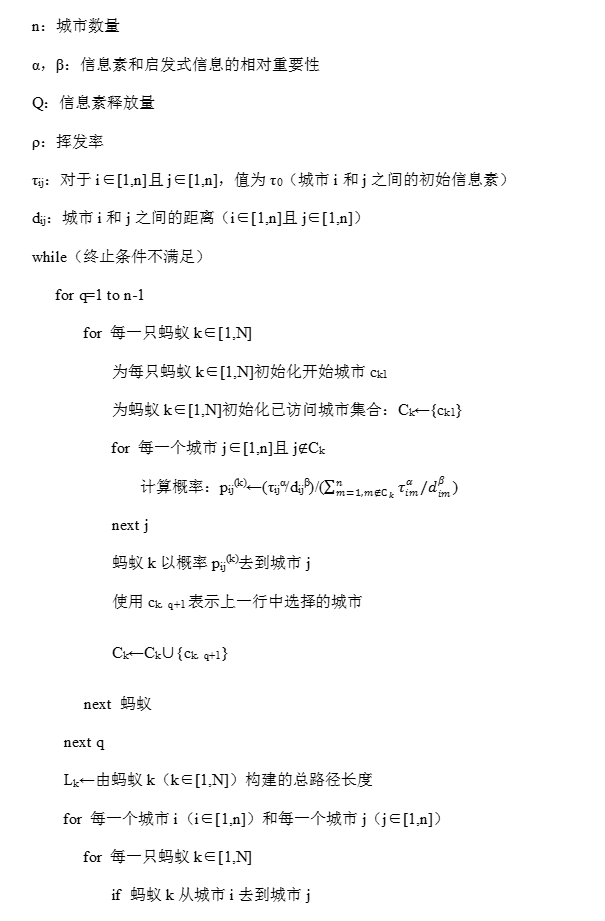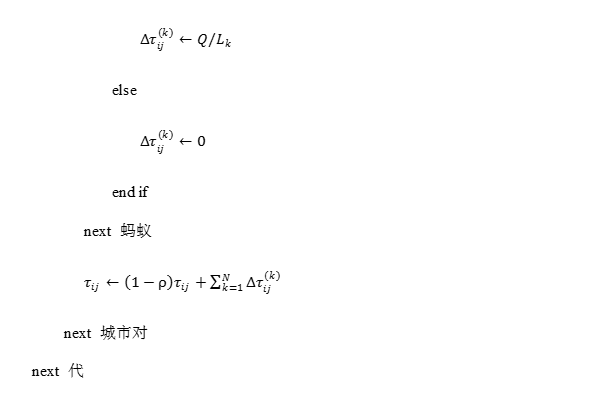从算法中可以看出每只蚂蚁从城市i去到城市j的概率与这些城市之间路径上信息素的数量成正比，与这些城市之间的距离成反比。比率α/β确定信息素信息相对于距离信息在决定去哪个城市旅行时的重要性。当一只蚂蚁从一个城市i移动到另一个城市j时，这条路径上的信息素的数量与蚂蚁解的质量成正比(也就是说，与蚂蚁的总旅行距离成反比)。
5.4 三种模型
5.4.1 蚁密系统模型
该模型中，一只蚂蚁经过路径(i，j)上释放的信息素量为常数Q，即\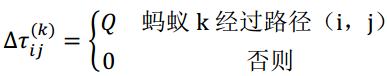5.4.2 蚁量系统模型
该模型中，一只蚂蚁经过路径(i，j)上释放的信息素量为常数Q/dij，即\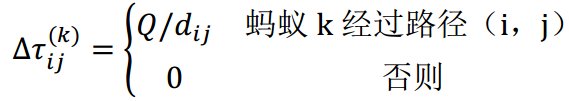\ 可以看出，在蚁量模型中，信息素轨迹强度的增加与dij成反比，因此段路径对蚂蚁更有吸引力。
5.4.3 蚁周系统模型
该模型与前两种模型的区别在于蚂蚁k完成一次循环后才会更新信息素，其更新公式如下：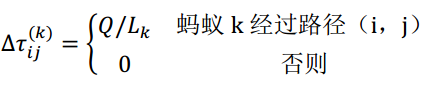\ 在蚁密模型和蚁量模型中，蚂蚁在建立方案的同时释放信息素，利用的是局部信息，而蚁周模型是在蚂蚁已经建立了完整的轨迹后再释放信息素，利用的是整体信息。
5.5 改进的蚁群优化算法
5.5.1 带精英策略的蚂蚁系统
带精英策略的蚂蚁系统(Ant System with elitist strategy，ASelite)，为了使当前的最优解在下一循环中对蚂蚁更有吸引力，在每次循环后给予最优解以额外的信息素，这个最优解就是全局最优解，找到该解的蚂蚁为精英蚂蚁，信息素更新公式如下：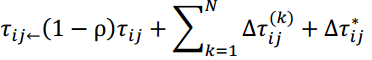\\\ δij(k)表示第k个蚂蚁经过ij，σ是精英解的个数。
5.5.2 基于优化排序的蚂蚁系统
和蚂蚁系统一样，带精英策略的蚂蚁系统有一个缺点：若在进化过程中，解的总质量提高了，解元素之间的差异减少了，导致选择概率的差异随之减小，使得搜索过程不会集中在到目前为止所找出的最优解附近，从而阻止了对更优解的进一步搜索。当路径长度变得非常接近，特别是当很多蚂蚁沿着局部极优的路径行进时，则对短路径的增强作用被削弱了。
受遗传算法中采用排序选择机制解决选择压力的启发，将排序的概念应用到蚂蚁系统中，就有了基于优先排序的蚂蚁系统(Rank-Bsed Version of Ant System，ASrank)。具体过程如下：当每只蚂蚁都生成一条路径后，蚂蚁按照路径长度排序(L1≤L2≤┈≤Lm)，蚂蚁对信息素轨迹更新的贡献根据该蚂蚁的排名µ的位次进行加权。此外只考虑w只最好的蚂蚁，σ为目前为止所找出的最优路径上轨迹贡献的权值，前σ-1只蚂蚁中的各只所经历的边获得一定量的信息素，其正比于该蚂蚁的排名位次。此外，到目前为止找出最优路径的蚂蚁所经过的边也将获得额外的信息素(这相当于带有精英策略的蚂蚁系统中精英蚂蚁的更新)，所以有如下的更新：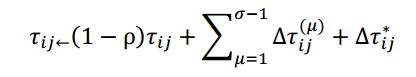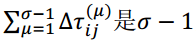是σ-1只蚂蚁根据排名对信息素轨迹的更新：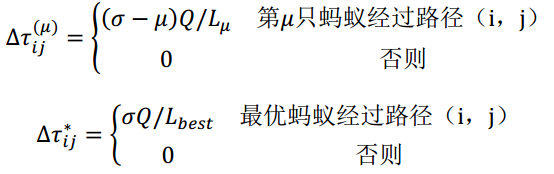5.5.3 蚁群系统
蚁群系统(Ant Colony System，ACS)是由Dorigo和Gambardella在1996年提出的，用于改善蚂蚁系统的性能。蚁群系统在蚂蚁系统的基础上主要做了三个方面的改进：\ (1)状态转移规则为更好更合理地利用新路径和利用关于问题的先验知识提供了方法。
蚁群系统使用双重决策：既可以利用关于问题的先验知识和以信息素形式存储的已经获得信息，又可以进行有向性的探索。通过调节参数q0，可以调节探索新路径的程度和是否使蚂蚁的搜索活动集中于最优解的空间邻域内。
(2)全局更新规则只应用于最优的蚂蚁路径上
在蚁群系统中，每次循环后只对最优蚂蚁经历的路径进行信息素的增强，其他路径由于挥发机制信息素会逐渐减少，这就增大了最优路径和最差路径在信息素上的差异，使得蚂蚁更倾向于选择最优路径中的边，从而使得其搜索行为能够很快地集中造最优路径附近，提高了算法的搜索效率。
(3)建立问题解决方案的过程中，应用局部信息素更新规则
蚂蚁在构造路径的同时进行局部更新，类似于蚁密和蚁量模型中的局部更新，而在每次循环后再对路径进行一次全局的更新。
5.5.3.1 蚁群系统状态转移规则
蚁群系统在构造候选解时使用了伪随机比例规则，位于节点i的蚂蚁通过下式确定选择下一个城市j的概率：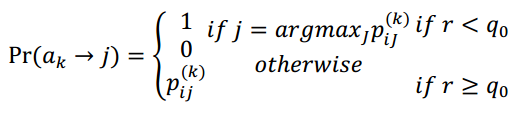\ r为[0,1]内均匀分布的随机数。
5.5.3.2 蚁群系统全局更新规则
在蚁群系统中，只有全局最优的蚂蚁才被允许释放信息素，这种选择以及伪随机比例规则，其目的都是为了使搜素过程更具有指导性：蚂蚁的搜索主要集中在当前循环为止所找出的最好路径的邻域内。全局更新如下：\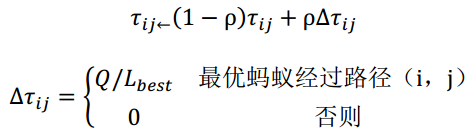5.5.3.3 蚁群系统局部更新规则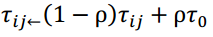\ 由实验发现，设置τ_0=(nL_nn )^(-1)可以产生好的结果，其中n是城市的数量，Lnn是由最近的邻域启发产生的一个路径长度。局部更新规则的应用使得相应的信息素轨迹减少，可以有效避免蚂蚁收敛到同一路径。
5.5.4 最大-最小蚂蚁系统
最大-最小蚂蚁系统(Max-min Ant System，MMAS)是对标准蚂蚁系统算法的一个简单修改[8, 9]。它有两个主要特点。首先，每一代最好的蚂蚁才能增加信息素，这就减少了开发并增加了对已知的开采。其次，信息素的量是上下有界的，这起到有相反的作用，也就是说，它增加了探索，因为即使是最糟糕的旅行也保留了非零数量的信息素，即使是最好的旅行也无法获得如此多的信息素，以至于完全控制了蚂蚁的决策。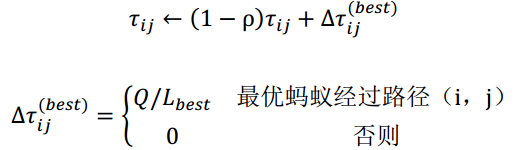若一条路径的信息素轨迹明显高于其他路径，停滞现象就会发生，因为在这种情况下蚂蚁更倾向于选择该路径，正反馈机制使得该路径的信息素进一步增强，从而蚂蚁将重复地建立同一个解，对搜索空间的探索停止。因此最大-最小蚂蚁系统对信息素轨迹的最大值和最小值分别施加了τ_min和τ_max限制，从而有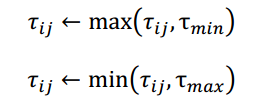5.5.5 最优-最差蚂蚁系统
鉴于蚂蚁系统搜索效率低和质量差的缺点，提出了一种最优-最差蚂蚁系统(Best-Worst Ant System，BWAS)。该算法的思想是对最优解进行更大限度的增强，而对最差解进行削弱，使得属于最优路径的边与属于最差路径的边之间的信息素差异进一步增大，从而使蚂蚁的搜索行为更集中于最优的附近。\ 该算法主要修改了蚁群系统中的全局更新，当所有蚂蚁完成一次循环后，增加对最差蚂蚁所经历路径的信息素的更新。若(i，j)为最差蚂蚁路径中的一条边，且不是最优蚂蚁路径中的边，则该路径的信息素按如下调整：\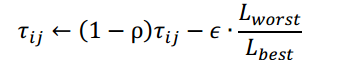5.6 代码
 %% 该函数用于演示基于蚁群算法的三维路径规划算法
%% 清空环境 clc clear
%% 数据初始化
%网格划分 LevelGrid=10; PortGrid=21;
%起点终点网格点 starty=10;starth=4; endy=8;endh=5; m=1; %算法参数 PopNumber=10; %种群个数 BestFitness=[]; %最佳个体
%初始信息素 pheromone=ones(21,21,21);
%% 初始搜索路径 [path,pheromone]=searchpath(PopNumber,LevelGrid,PortGrid,pheromone, ... HeightData,starty,starth,endy,endh); fitness=CacuFit(path); %适应度计算 [bestfitness,bestindex]=min(fitness); %最佳适应度 bestpath=path(bestindex,:); %最佳路径 BestFitness=[BestFitness;bestfitness]; %适应度值记录
%% 信息素更新 rou=0.2; cfit=100/bestfitness; for i=2:PortGrid-1 pheromone(i,bestpath(i2-1),bestpath(i2))= ... (1-rou)pheromone(i,bestpath(i2-1),bestpath(i2))+roucfit; end
%% 循环寻找最优路径 for kk=1:100
%% 路径搜索
[path,pheromone]=searchpath(PopNumber,LevelGrid,PortGrid,...
pheromone,HeightData,starty,starth,endy,endh);

%% 适应度值计算更新
fitness=CacuFit(path);
[newbestfitness,newbestindex]=min(fitness);
if newbestfitness<bestfitness
bestfitness=newbestfitness;
bestpath=path(newbestindex,:);
end
BestFitness=[BestFitness;bestfitness];

%% 更新信息素
cfit=100/bestfitness;
for i=2:PortGrid-1
pheromone(i,bestpath(i*2-1),bestpath(i*2))=(1-rou)* ...
pheromone(i,bestpath(i*2-1),bestpath(i*2))+rou*cfit;
end
end
%% 最佳路径 for i=1:21 a(i,1)=bestpath(i2-1); a(i,2)=bestpath(i2); end figure(1) x=1:21; y=1:21; [x1,y1]=meshgrid(x,y); mesh(x1,y1,HeightData) axis([1,21,1,21,0,2000]) hold on k=1:21; plot3(k(1)',a(1,1)',a(1,2)'200,'--o','LineWidth',2,... 'MarkerEdgeColor','k',... 'MarkerFaceColor','g',... 'MarkerSize',10) plot3(k(21)',a(21,1)',a(21,2)'200,'--o','LineWidth',2,... 'MarkerEdgeColor','k',... 'MarkerFaceColor','g',... 'MarkerSize',10) text(k(1)',a(1,1)',a(1,2)'200,'S'); text(k(21)',a(21,1)',a(21,2)'200,'T'); xlabel('km','fontsize',12); ylabel('km','fontsize',12); zlabel('m','fontsize',12); title('三维路径规划空间','fontsize',12) set(gcf, 'Renderer', 'ZBuffer') hold on plot3(k',a(:,1)',a(:,2)'*200,'--o')
%% 适应度变化 figure(2) plot(BestFitness) title('最佳个体适应度变化趋势') xlabel('迭代次数') ylabel('适应度值')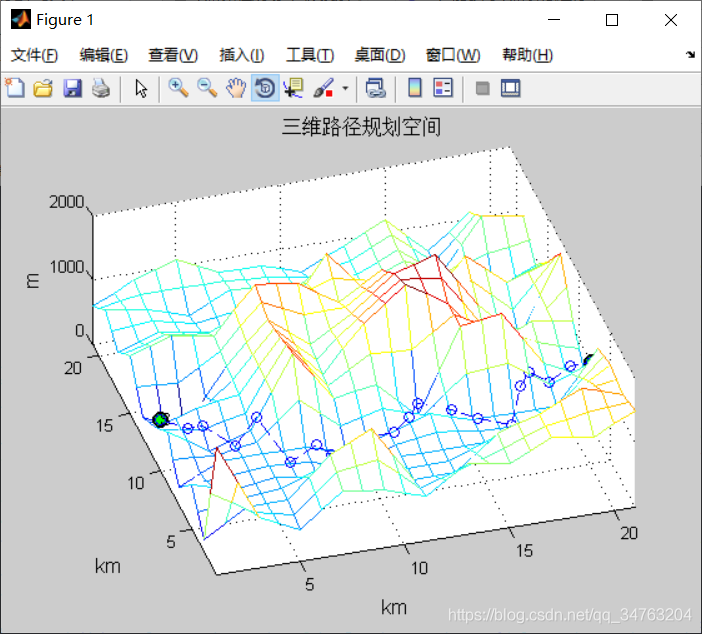展开全文算法 大数据 python 机器学习 人工智能
• 路径规划】基于粒子群的无人机三维路径规划matlab源码
• 路径问题而言，在具体实施任务时仅靠操作员手中的遥控器控制无人飞行器执行相应的工作，可能会对操作员心理以及技术提出极高的要求，为了避免个人操作失误，进而造成飞行器损坏的危险，一种解决问题的方法就是对...
一、无人机简介
0 引言 随着现代技术的发展，飞行器种类不断变多，应用也日趋专一化、完善化，如专门用作植保的大疆PS-X625无人机，用作街景拍摄与监控巡察的宝鸡行翼航空科技的X8无人机，以及用作水下救援的白鲨MIX水下无人机等，决定飞行器性能主要是内部的飞控系统和外部的路径规划问题。就路径问题而言，在具体实施任务时仅靠操作员手中的遥控器控制无人飞行器执行相应的工作，可能会对操作员心理以及技术提出极高的要求，为了避免个人操作失误，进而造成飞行器损坏的危险，一种解决问题的方法就是对飞行器进行航迹规划。 飞行器的测量精度，航迹路径的合理规划，飞行器工作时的稳定性、安全性等这些变化对飞行器的综合控制系统要求越来越高。无人机航路规划是为了保证无人机完成特定的飞行任务，并且能够在完成任务的过程中躲避各种障碍、威胁区域而设计出最优航迹路线的问题。常见的航迹规划算法如图1所示。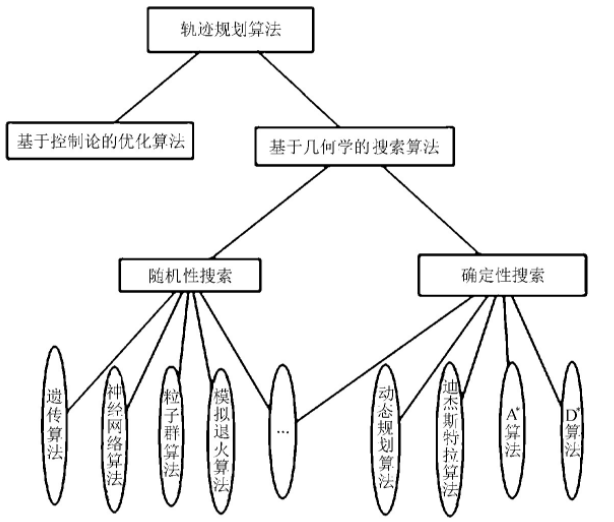图1 常见路径规划算法 文中主要对无人机巡航阶段的航迹规划进行研究，假设无人机在飞行中维持高度与速度不变，那么航迹规划成为一个二维平面的规划问题。在航迹规划算法中,A算法计算简单，容易实现。在改进A算法基础上，提出一种新的、易于理解的改进A算法的无人机航迹规划方法。传统A算法将规划区域栅格化，节点扩展只限于栅格线的交叉点，在栅格线的交叉点与交叉点之间往往存在一定角度的两个运动方向。将存在角度的两段路径无限放大、细化，然后分别用两段上的相应路径规划点作为切点，找到相对应的组成内切圆的圆心，然后作弧，并求出相对应的两切点之间的弧所对应的圆心角，根据下式计算出弧线的长度式中：R———内切圆的半径； α———切点之间弧线对应的圆心角。
二、蝙蝠优化算法（BA）简介
蝙蝠算法( BA) 是 Yang 教授于 2010 年基于群体智能提出的启发式搜索算法,是一种搜索全局最优解的有效方法。该算法是一种基于迭代的优化技术,初始化为一组随机解,然后 通过迭代搜寻最优解,且在最优解周围通过随机飞行产生局部新解,加强了局部搜索。与其他算法相比,BA 在准确性和有效性方面远优于其他算法,且没有许多参数要进行调整。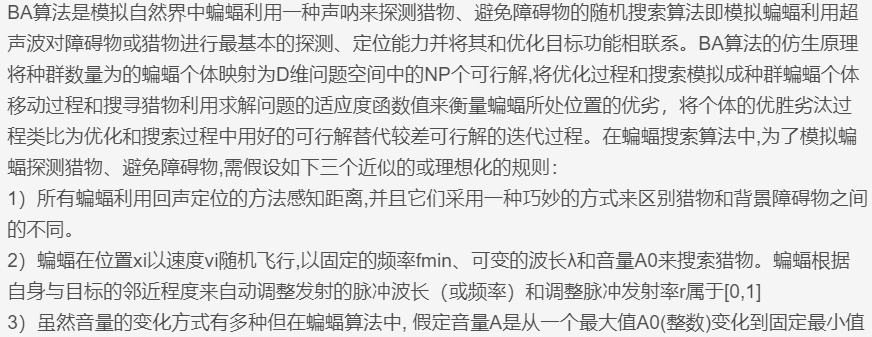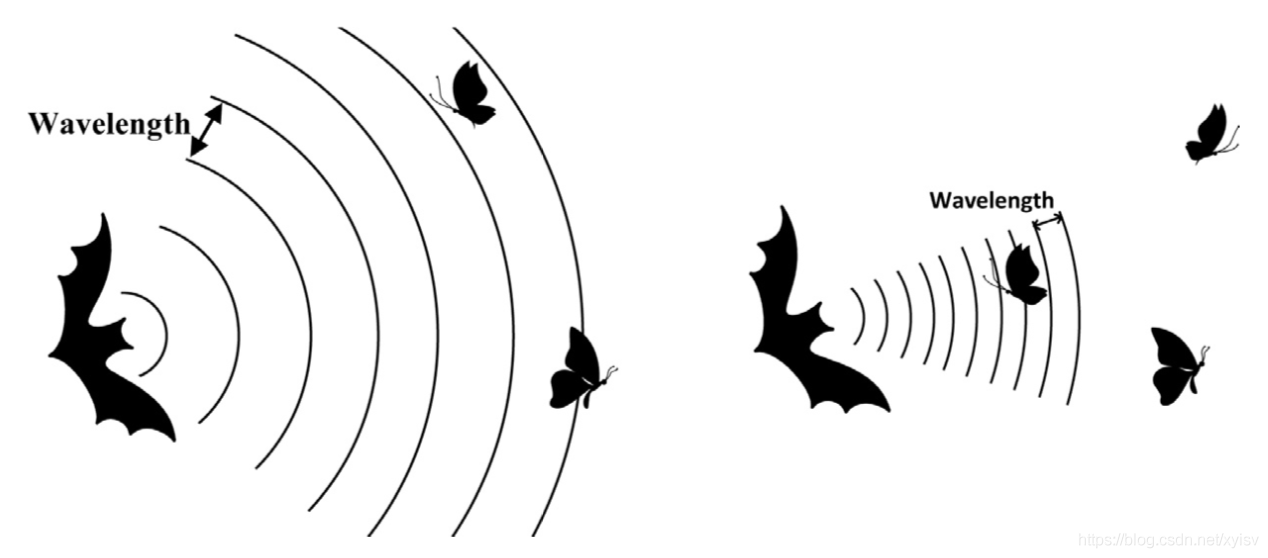三、差分进化算法简介
1 前言
在遗传、选择和变异的作用下，自然界生物体优胜劣汰，不断由低级向高级进化和发展。人们注意到，适者生存的进化规律可以模式化，从而构成一些优化算法；近年来发展的进化计算类算法受到了广泛的关注。 差分进化算法(Differential Evolution， DE) 是一种新兴的进化计算技术 。它是由S torn等人于1995年提出的， 其最初的设想是用于解决切比雪夫多项式问题，后来发现它也是解决复杂优化问题的有 效技术。 差分进化算法是基于群体智能理论的优化算法，是通过群体内个体间的合作与竞争而产生的智能优化搜索算法。但相比于进化计算，它保留了基于种群的全局搜索策略，采用实数编码、基于差分的简单 变异操作和“一对一”的竞争生存策略，降低了进化计算操作的复杂性。同时，差分进化算法特有的记忆能力使其可以动态跟踪当前的搜索情况，以调整其搜索策略，它具有较强的全局收敛能力和稳健性， 且不需要借助问题的特征信息，适用于求解一些利用常规的数学规划方法很难求解甚至无法求解的复杂优化问题[2-5]。因此，差分进化算法作为一种高效的并行搜索算法，对其进行理论和应用研究具有重要的学术意义和工程价值。 目前，差分进化算法已经在许多领域得到了应用，如人工神经元网络、电力、机械设计、机器人、信号处理、生物信息、经济学、现代农业和运筹学等。然而，尽管差分进化算法获得了广泛研究，但相 对于其他进化算法而言，其研究成果相当分散，缺乏系统性，尤其在理论方面还没有重大突破。
2 差分进化算法理论 2.1差分进化算法原理 差分进化算法是一种随机的启发式搜索算法，简单易用，有较强的鲁棒性和全局寻优能力。它从数学角度看是一种随机搜索算法，从工程角度看是一种自适应的迭代寻优过程。除了具有较好的收敛性外，差分进化算法非常易于理解与执行，它只包含不多的几个控制参数，并且在整个迭代过程中，这些参数的值可以保持不变。差分进化算法是一种自组织最小化方法，用户只需很少的输入。它的关键思想与传统进化方法不同：传统方法是用预先确定的概率分布函数决定向量扰动；而差分进化算法的自组织程序利用种群中两个随机选择的不同向量来干扰一个现有向量，种群中的每一个向量都要进行干扰。差分进化算法利用一个向量种群，其中种群向量的随机扰动可独立进行，因此是并行的。如果新向量对应函数值的代价比它们的前辈代价小，它们将取代前辈向量。 同其他进化算法一样，差分进化算法也是对候选解的种群进行操作，但其种群繁殖方案与其他进化算法不同：它通过把种群中两个成员之间的加权差向量加到第三个成员上来产生新的参数向量，该操作 称为“变异”； 然后将变异向量的参数与另外预先确定的目标向量参数按一定规则混合来产生试验量，该操作称为“交叉”；最后，若试验向量的代价函数比目标向量的代价函数低，试验向量就在下一代中代替目标向量，该操作称为“选择”。种群中所有成员必须当作目标向量进行一次这样的操作，以便在下一代中出现相同个数竞争者。 在进化过程中对每一代的最佳参数向量都进行评价，以记录最小化过程。这样利用随机偏差扰动产生新个体的方式，可以获得一个收敛性非常好的结果，引导搜索过程向全局最优解逼近[6-7]。
2.2差分进化算法的特点 差分进化算法从提出到现在，在短短二十几年内人们对其进行了广泛的研究并取得了成功的应用。该算法主要有如下特点： (1)结构简单，容易使用。差分进化算法主要通过差分变异算子来进行遗传操作，由于该算子只涉及向量的加减运算，因此很容易实现；该算法采用概率转移规则，不需要确定性的规则。此外，差分进化算法的控制参数少，这些参数对算法性能的影响已经得到一定的研究，并得出了一些指导性的建议，因而可以方便使用人员根据问题选择较优的参数设置。 (2)性能优越。差分进化算法具有较好的可靠性、高效性和鲁棒性，对于大空间、非线性和不可求导的连续问题，其求解效率比其他进化方法好，而且很多学者还在对差分进化算法继续改良，以不断提高其性能。 (3)自适应性。差分进化算法的差分变异算子可以是固定常数，也可以具有变异步长和搜索方向自适应的能力，根据不同目标函数进行自动调整，从而提高搜索质量。 (4)差分进化算法具有内在的并行性，可协同搜索，具有利用个体局部信息和群体全局信息指导算法进一步搜索的能力。在同样精度要求下，差分进化算法具有更快的收敛速度。 (5)算法通用，可直接对结构对象进行操作，不依赖于问题信息，不存在对目标函数的限定。差分进化算法操作十分简单，易于编程实现，尤其利于求解高维的函数优化问题。
3 差分进化算法种类 3.1基本差分进化算法 基本差分进化算法的操作程序如下： (1)初始化； (2)变异； (3)交叉； (4)选择； (5)边界条件处理。 初始化 差分进化算法利用NP个维数为D的实数值参数向量，将它们作为每 一代的种群，每个个体表示为：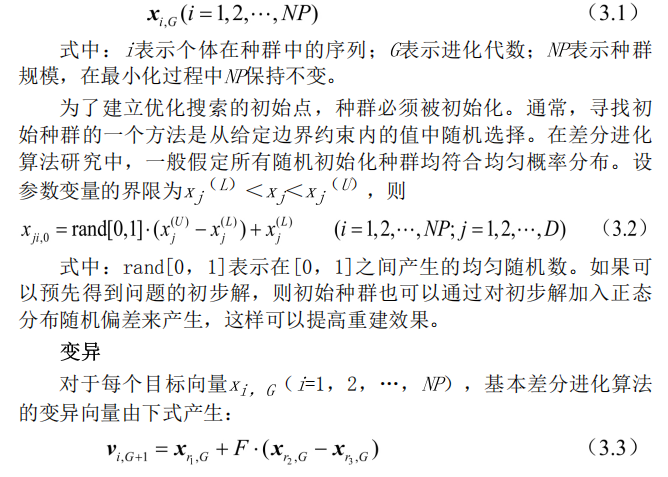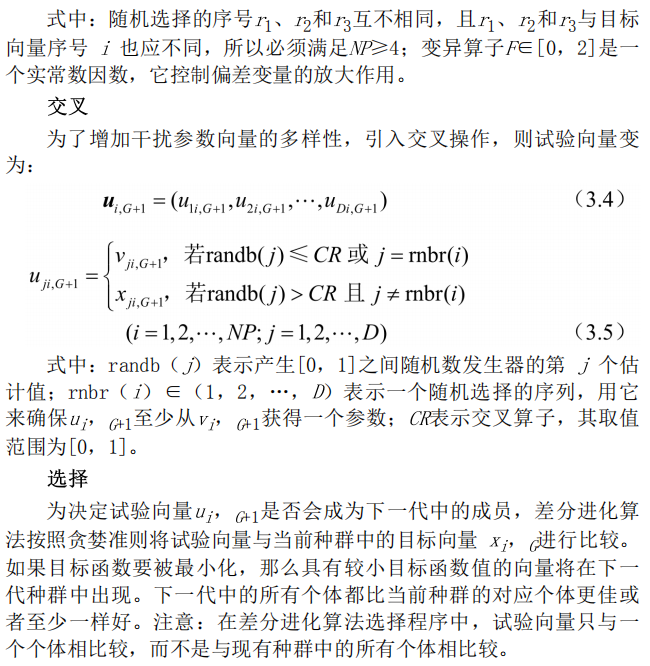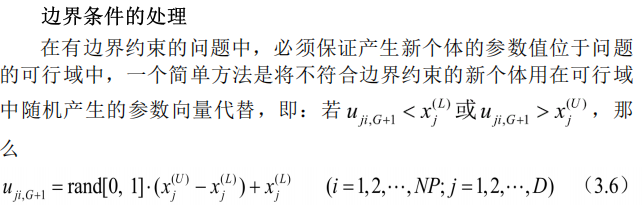另外一个方法是进行边界吸收处理，即将超过边界约束的个体值设置为临近的边界值。
3.2差分进化算法的其他形式 上面阐述的是最基本的差分进化算法操作程序，实际应用中还发展了差分进化算法的几个变形形式，用符号DE/x/y/z加以区分，其中：x限定当前被变异的向量是“随机的”或“最佳的”；y是所利用的差向量的个数；z指示交叉程序的操作方法。前面叙述的交叉操作表示为“bin”。利用这个表示方法， 基本差分进化算法策略可描述为DE/rand/1/bin。 还有其他形式[5，如：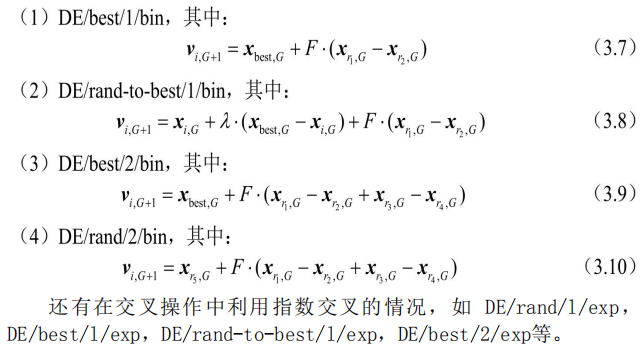3.3改进的差分进化算法 自适应差分进化算法 作为一种高效的并行优化算法，差分进化算法发展很快，出现了很多改进的差分进化算法。下面介绍一种具有自适应算子的差分进化算法.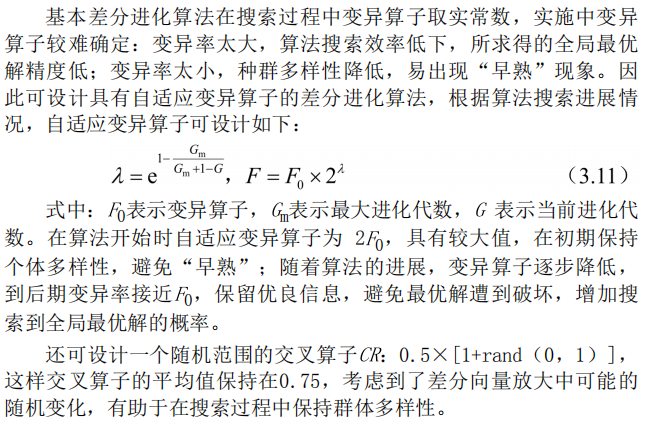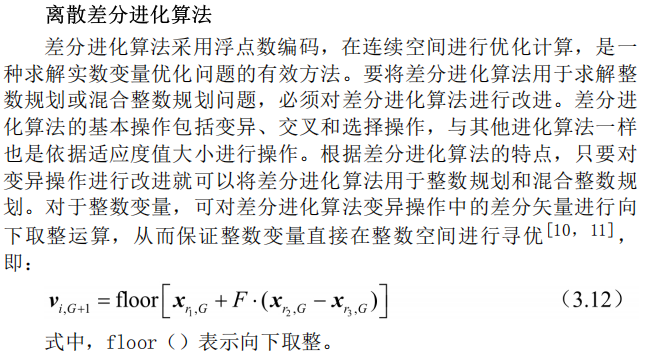4差分进化算法流程 差分进化算法采用实数编码、基于差分的简单变异操作和“一对一”的竞争生存策略，其具体步骤如下： (1)确定差分进化算法的控制参数和所要采用的具体策略。差分进化算法的控制参数包括：种群数量、变异算子、交叉算子、最大进化代数、终止条件等。 (2)随机产生初始种群，进化代数k=1。 (3)对初始种群进行评价，即计算初始种群中每个个体的目标函数值。 (4)判断是否达到终止条件或达到最大进化代数：若是，则进化终止，将此时的最佳个体作为解输出；否则，继续下一步操作。 (5)进行变异操作和交叉操作，对边界条件进行处理，得到临时种群。 (6)对临时种群进行评价，计算临时种群中每个个体的目标函数值。 (7)对临时种群中的个体和原种群中对应的个体，进行“一对-”的选择操作，得到新种群。 (8)进化代数k=k+1，转步骤(4)。 差分进化算法运算流程如图3.1所示。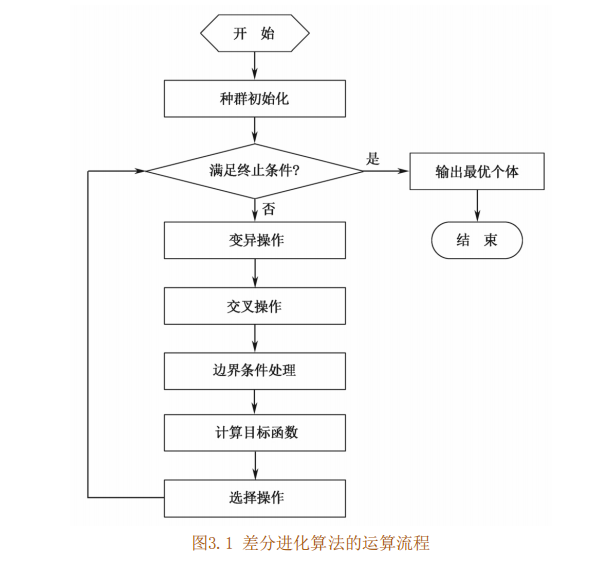5关键参数的说明 控制参数对一个全局优化算法的影响是很大的，差分进化算法的控制变量选择也有一些经验规则。
种群数量NP 一般情况下，种群的规模AP越大，其中的个体就越多，种群的多样性也就越好，寻优的能力也就越强，但也因此增加了计算的难度。所以，NP不能无限取大。根据经验，种群数量NP的合理选择在5D~ 10D之间，必须满足NP≥4，以确保差分进化算法具有足够的不同的变异向量。
变异算子F 变异算子FE[0，2]是一个实常数因数，它决定偏差向量的放大比例。变异算子熨小，则可能造成算法“早熟”。随着/值的增大，防止算法陷入局部最优的能力增强，但当F>1时，想要算法快速收敛到最优值会变得十分不易；这是由于当差分向量的扰动大于两个个体之间的距离时，种群的收敛性会变得很差。目前的研究表明，F小于0.4和大于1的值仅偶尔有效，/=0.5通常是一个较好的初始选择。若种 群过早收敛，那么F或NP应该增大。
交叉算子CR 交叉算子CR是一个范围在[0，1]内的实数，它控制着一个试验向量参数来自于随机选择的变异向量而不是原来向量的概率。交叉算子CK越大，发生交叉的可能性就越大。CR的一个较好的选择是0.1，但 较大的CK通常会加速收敛，为了看看是否可能获得一个快速解，可以先尝试CR=0.9或CF=1.0.
最大进化代数G 最大进化代数6是表示差分进化算法运行结束条件的一个参数，表示差分进化算法运行到指定的进化代数之后就停止运行，并将当前群体中的最佳个体作为所求问题的最优解输出。一般，6取100~500。
终止条件 除最大进化代数可作为差分进化算法的终止条件外，还可以增加其他判定准则。一般当目标函数值小于阈值时程序终止，阈值常选为10-6。上述参数中，F、CR与NP一样，在搜索过程中是常数，一般F和CR影响搜索过程的收敛速度和稳健性，它们的优化值不仅依赖于目标函数的特性，还与NP有关。通常可通过对不同值做一些试验之后，利用试验和结果误差找到F、CR和NP的合适值。
四、部分源代码
clear all;
close all;
clc;
tic
%%
global k;
global Threat_kind;
global d;
global Threat_center;
M=60; %种群规模

F1=0.7;
F2=0.8;
F3=0.9;

CR=0.5;
D=20; %函数优化的维度
NCmax=100;%迭代次数
start=[0;0]; %起始点坐标

aim=[80;100];  %执行任务终点
distance=sqrt((start(1)-aim(1))^2+(start(2)-aim(2))^2); %起始点和终点之间的距离

a=(aim(1)-start(1))/distance;%起始点终点连线与水平线之间夹角的余弦值
b=(aim(2)-start(2))/distance;%起始点终点连线与水平线之间夹角的正弦值

Threat_center=[10 30 90 20 50 65 60 30 60 75;50 80 80 20 55 38 80 42 10 65];%威胁中心
Threat_radius=[10 10 10 9 10 10 10 8 10 8];%威胁半径
Threat_kind=[8 4 10 6 7 6 7 5 6 8]; %威胁代价权值

k=0.9;%威胁代价权值

Path1=zeros(M,D);
Path2=zeros(M,D);
Path3=zeros(M,D);

cross_Path1=zeros(M,D);
cross_Path2=zeros(M,D);
cross_Path3=zeros(M,D);

mutate_Path1=zeros(M,D);
mutate_Path2=zeros(M,D);
mutate_Path3=zeros(M,D);

mutate_Path_glob1=zeros(M,D);
mutate_Path_glob2=zeros(M,D);
mutate_Path_glob3=zeros(M,D);

mutate_Path_loc1=zeros(M,D);
mutate_Path_loc2=zeros(M,D);
mutate_Path_loc3=zeros(M,D);

uavroute1=zeros(NCmax,D);
uavroute2=zeros(NCmax,D);
uavroute3=zeros(NCmax,D);

Path_bestmem=zeros(1,D);
Path_bestmemit1=zeros(1,D);
Path_bestmemit2=zeros(1,D);
Path_bestmemit3=zeros(1,D);
Path_bm1=zeros(M,D);
Path_bm2=zeros(M,D);
Path_bm3=zeros(M,D);
Best_solution1=zeros(1,D);
Best_solution2=zeros(1,D);
Best_solution3=zeros(1,D);
Fitness1=ones(1,M);
Fitness2=ones(1,M);
Fitness3=ones(1,M);
iteration_fitness1=zeros(1,NCmax);
iteration_fitness2=zeros(1,NCmax);
iteration_fitness3=zeros(1,NCmax);

%% 坐标系变换
size=length(Threat_kind);
for i=1:size
Threat_transform(1,i)=a*(Threat_center(1,i)-start(1))+b*(Threat_center(2,i)-start(2));
Threat_transform(2,i)=-b*(Threat_center(1,i)-start(1))+a*(Threat_center(2,i)-start(2));
end

start_transform=[0 0]; %起始点坐标转换
aim_transform(1)=a*(aim(1)-start(1))+b*(aim(2)-start(2));
aim_transform(2)=-b*(aim(1)-start(1))+a*(aim(2)-start(2)); %目标点坐标转换

%% 初始化航路
d=aim_transform(1)/(D+1); %旋转坐标系的横坐标间隔
for i=1:M
Path1(i,1)=(rand-0.45)*20;
Path2(i,1)=(rand-0.55)*20;
Path3(i,1)=(rand-0.5)*20;
for j=2:D
Path1(i,j)=Path1(i,j-1)+(rand-0.45)*10;
Path2(i,j)=Path2(i,j-1)+(rand-0.55)*10;
Path3(i,j)=Path3(i,j-1)+(rand-0.5)*10;
end %初始化横坐标
end
tic
%% 迭代计算
for NC=1:NCmax
I_best_index1=1;
I_best_index2=1;
I_best_index3=1;
I_strategy=3;
NDE_an1=1;
NDE_an2=2;
Nde=NC/NCmax;
for i=1:M
r1=ceil(M*rand);
r2=ceil(M*rand);
r3=ceil(M*rand);
while(r1==r2||r1==r3||r2==r3)
r1=ceil(M*rand);
r2=ceil(M*rand);
r3=ceil(M*rand);
end               %选取不同的r1,r2,r3，且不等于i

if(NC>=2&&I_strategy==1)
mutate_Path1(i,:)=Path_bm1(i,:)+F1.*(Path1(r1,:)-Path1(r2,:));
mutate_Path2(i,:)=Path_bm1(i,:)+F2.*(Path2(r1,:)-Path2(r2,:));
mutate_Path3(i,:)=Path_bm1(i,:)+F3.*(Path3(r1,:)-Path3(r2,:));
elseif (NC>=2&&I_strategy==2)
mutate_Path1(i,:)=Path1(i,:)+F1*(Path_bm1(i,:)-Path1(i,:))+F1*(Path1(r1,:)-Path1(r2,:));
mutate_Path2(i,:)=Path2(i,:)+F2*(Path_bm1(i,:)-Path2(i,:))+F2*(Path2(r1,:)-Path2(r2,:));
mutate_Path3(i,:)=Path3(i,:)+F3*(Path_bm1(i,:)-Path3(i,:))+F3*(Path3(r1,:)-Path3(r2,:));
elseif(NC>=2&&I_strategy==3&&i>3)
FVr_Nind=randsrc(1,6,[i-3,i-2,i-1,i+1,i+2,i+3]);
NDE_an1=rem(FVr_Nind(fix(rand(1)*6)+1),M);
NDE_an2=rem(FVr_Nind(fix(rand(1)*6)+1),M);
while (NDE_an2==NDE_an1||NDE_an1==0||NDE_an2==0)

end

mutate_Path_glob1(i,:)=Path_bm1(i,:)+(Path_bm1(i,:)-Path1(i,:)).*((1-0.9999)*rand(1,D)+F1)+(Path1(r1,:)-Path1(r2,:)).*((1-0.9999)*rand(1,D)+F1);
mutate_Path_loc1(i,:)=Path1(i,:)+F1*(Path_bm1(i,:)-Path1(i,:))+F1*(Path1(NDE_an1,:)-Path1(NDE_an2,:));
mutate_Path1(i,:)=Nde*mutate_Path_glob1(i,:)+(1-Nde)*mutate_Path_loc1(i,:);

mutate_Path_glob2(i,:)=Path_bm1(i,:)+(Path_bm1(i,:)-Path2(i,:)).*((1-0.9999)*rand(1,D)+F2)+(Path2(r1,:)-Path2(r2,:)).*((1-0.9999)*rand(1,D)+F2);
mutate_Path_loc2(i,:)=Path2(i,:)+F2*(Path_bm1(i,:)-Path2(i,:))+F2*(Path2(NDE_an1,:)-Path2(NDE_an2,:));
mutate_Path2(i,:)=Nde*mutate_Path_glob2(i,:)+(1-Nde)*mutate_Path_loc2(i,:);

mutate_Path_glob3(i,:)=Path_bm1(i,:)+(Path_bm1(i,:)-Path3(i,:)).*((1-0.9999)*rand(1,D)+F3)+(Path3(r1,:)-Path3(r2,:)).*((1-0.9999)*rand(1,D)+F3);
mutate_Path_loc3(i,:)=Path3(i,:)+F3*(Path_bm1(i,:)-Path3(i,:))+F3*(Path3(NDE_an1,:)-Path3(NDE_an2,:));
mutate_Path3(i,:)=Nde*mutate_Path_glob3(i,:)+(1-Nde)*mutate_Path_loc3(i,:);
else
mutate_Path1(i,:)=Path1(r1,:)+F1.*(Path1(r2,:)-Path1(r3,:));
mutate_Path2(i,:)=Path2(r1,:)+F2.*(Path2(r2,:)-Path2(r3,:));
mutate_Path3(i,:)=Path3(r1,:)+F3.*(Path3(r2,:)-Path3(r3,:));
end
randr=ceil(D*rand);
for j=1:D
if j==randr||rand<=CR
cross_Path1(i,j)=mutate_Path1(i,j);
cross_Path2(i,j)=mutate_Path2(i,j);
cross_Path3(i,j)=mutate_Path3(i,j);
else
cross_Path1(i,j)=Path1(i,j);
cross_Path2(i,j)=Path2(i,j);
cross_Path3(i,j)=Path3(i,j);
end

end         %产生交叉个体

new_threat1=Threat_count(aim_transform(),cross_Path1(i,:),Threat_transform);
formal_threat1=Threat_count(aim_transform(),Path1(i,:),Threat_transform);
new_threat2=Threat_count(aim_transform(),cross_Path2(i,:),Threat_transform);
formal_threat2=Threat_count(aim_transform(),Path2(i,:),Threat_transform);
new_threat3=Threat_count(aim_transform(),cross_Path3(i,:),Threat_transform);
formal_threat3=Threat_count(aim_transform(),Path3(i,:),Threat_transform);
if new_threat1<=formal_threat1
Fitness1(i)=new_threat1;
Path1(i,:)=cross_Path1(i,:);
else
Fitness1(i)=formal_threat1;
Path1(i,:)=Path1(i,:);
end
if(Fitness1(i)==min(Fitness1))
I_best_index1=i;
end

if new_threat2<=formal_threat2
Fitness1(i)=new_threat2;
Path2(i,:)=cross_Path2(i,:);
else
Fitness2(i)=formal_threat2;
Path2(i,:)=Path2(i,:);
end
if(Fitness2(i)==min(Fitness2))
I_best_index2=i;
end

if new_threat3<=formal_threat3
Fitness3(i)=new_threat3;
Path3(i,:)=cross_Path3(i,:);
else

if(Fitness3(i)==min(Fitness3))
I_best_index3=i;
end

end

[iteration_fitness1(NC),flag1]=min(Fitness1);
[iteration_fitness2(NC),flag2]=min(Fitness2);
[iteration_fitness3(NC),flag3]=min(Fitness3);

uavroute1(NC,:)=Path1(flag1,:);
uavroute2(NC,:)=Path2(flag2,:);
uavroute3(NC,:)=Path3(flag3,:);

fprintf('NC=%d ObjVal=%g\n',NC,iteration_fitness1(NC));
fprintf('NC=%d ObjVal=%g\n',NC,iteration_fitness2(NC));
fprintf('NC=%d ObjVal=%g\n',NC,iteration_fitness3(NC));

iteration_fitness1(iteration_fitness1==1)=50;
iteration_fitness2(iteration_fitness2==1)=50;
iteration_fitness3(iteration_fitness3==1)=50;

Path_bestmemit1=Path1(I_best_index1,:);
Path_bestmemit2=Path2(I_best_index2,:);
Path_bestmemit3=Path3(I_best_index3,:);
for p=1:M
Path_bm1(p,:)=Path_bestmemit1;
Path_bm2(p,:)=Path_bestmemit2;
Path_bm3(p,:)=Path_bestmemit3;
end
end

[Best_fitness1,flag1]=min(iteration_fitness1);
[Best_fitness2,flag2]=min(iteration_fitness2);
[Best_fitness3,flag3]=min(iteration_fitness3);
Best_solution1=uavroute1(flag1,:);
Best_solution2=uavroute2(flag2,:);
Best_solution3=uavroute3(flag3,:);

for i=1:length(Best_solution1)
BestPath1(1,i+1)=a*i*d-b*Best_solution1(i)+start(1);
BestPath1(2,i+1)=b*i*d+a*Best_solution1(i)+start(2);
%     BestPath1(BestPath1<0)=5;
end
for i=1:length(Best_solution2)
BestPath2(1,i+1)=a*i*d-b*Best_solution2(i)+start(1);
BestPath2(2,i+1)=b*i*d+a*Best_solution2(i)+start(2);
%     BestPath2(BestPath2<0)=6;
end
for i=1:length(Best_solution3)
BestPath3(1,i+1)=a*i*d-b*Best_solution3(i)+start(1);
BestPath3(2,i+1)=b*i*d+a*Best_solution3(i)+start(2);
%     BestPath3(BestPath3<0)=4;
end
BestPath1(1,i+2)=aim(1);
BestPath1(2,i+2)=aim(2);
BestPath2(1,i+2)=aim(1);
BestPath2(2,i+2)=aim(2);
BestPath3(1,i+2)=aim(1);
BestPath3(2,i+2)=aim(2);

figure (1);
plot(BestPath1(1,:),BestPath1(2,:),'k*--','LineWidth',2);

hold on;
plot(BestPath2(1,:),BestPath2(2,:),'bo--','LineWidth',2);

hold on;
plot(BestPath3(1,:),BestPath3(2,:),'rx--','LineWidth',2);

hold on;
legend('CPFIBA','DEBA','BA');

% hold on;
% plot(iteration_fitness1,'k-');
% plot(iteration_fitness2,'b*');
% plot(iteration_fitness3,'r+');

% title('the objective function value convergence curve');
% xlabel('the number of iterations n');
% ylabel('the value of objective function ObjVal');
% hold off;

% hold on;
% plot(iteration_fitness1,'k-');
% plot(iteration_fitness2,'b*');
% plot(iteration_fitness3,'r+');
%
% title('the objective function value convergence curve');
% xlabel('the number of iterations n');
% ylabel('the value of objective function ObjVal');
hold off;

toc


五、运行结果六、matlab版本及参考文献
1 matlab版本 2014a
2 参考文献  包子阳,余继周,杨杉.智能优化算法及其MATLAB实例（第2版）[M].电子工业出版社，2016. 张岩,吴水根.MATLAB优化算法源代码[M].清华大学出版社，2017. 巫茜,罗金彪,顾晓群,曾青.基于改进PSO的无人机三维航迹规划优化算法[J].兵器装备工程学报. 2021,42(08) 邓叶,姜香菊.基于改进人工势场法的四旋翼无人机航迹规划算法[J].传感器与微系统. 2021,40(07) 马云红,张恒,齐乐融,贺建良.基于改进A*算法的三维无人机路径规划[J].电光与控制. 2019,26(10) 焦阳.基于改进蚁群算法的无人机三维路径规划研究[J].舰船电子工程. 2019,39(03)
展开全文算法
• 1 简介 2 部分代码 clear all close all clc syms x1 syms x2 grid on hold on axis equal N = 4;...[pos,obst,target,dist_matrix,lambda,sec_dist,mu,Cr,d,...
1 简介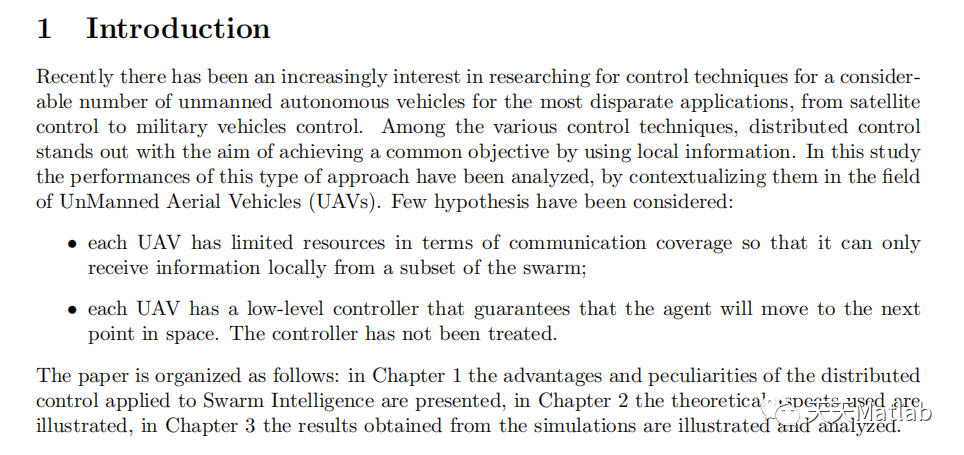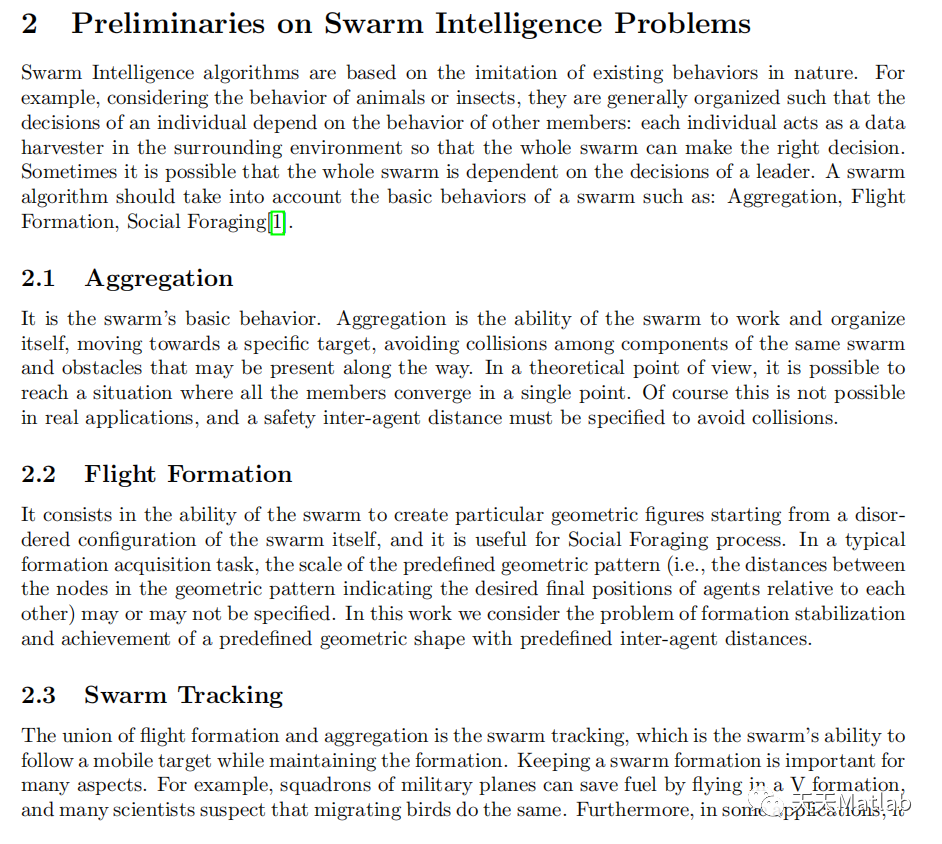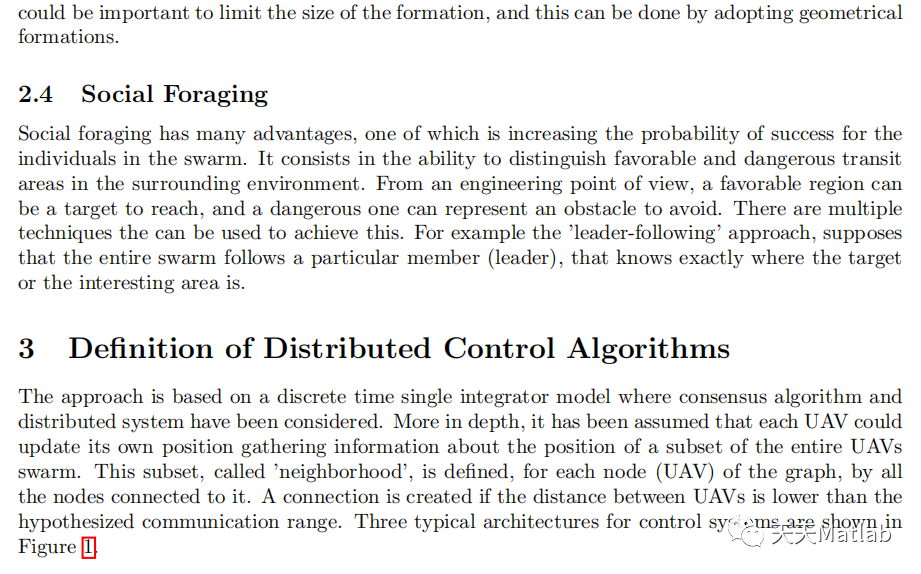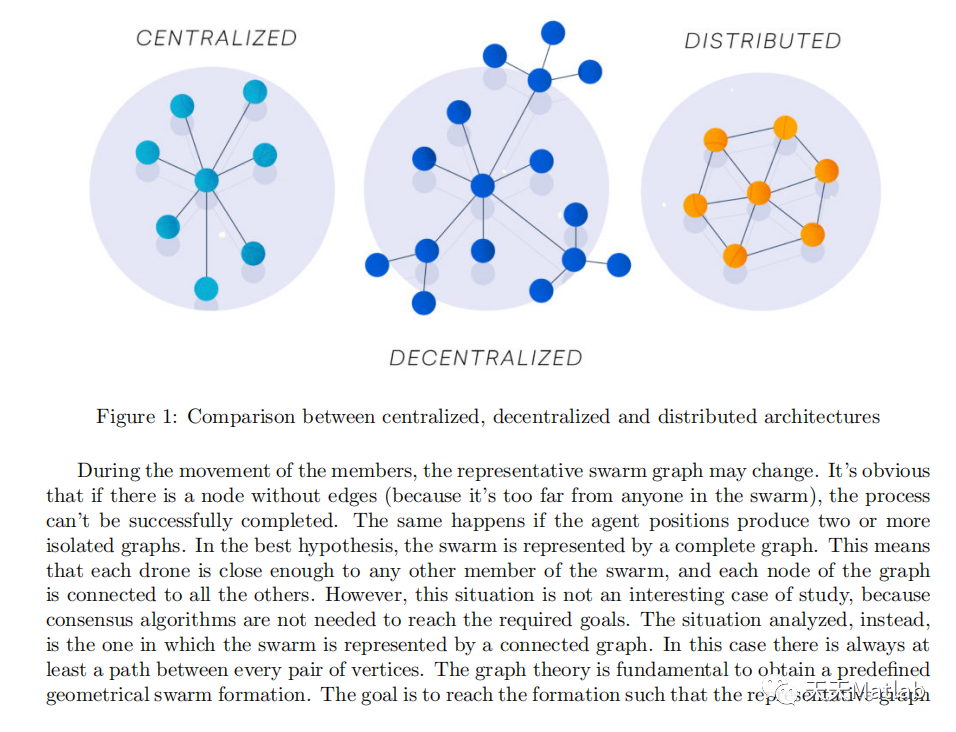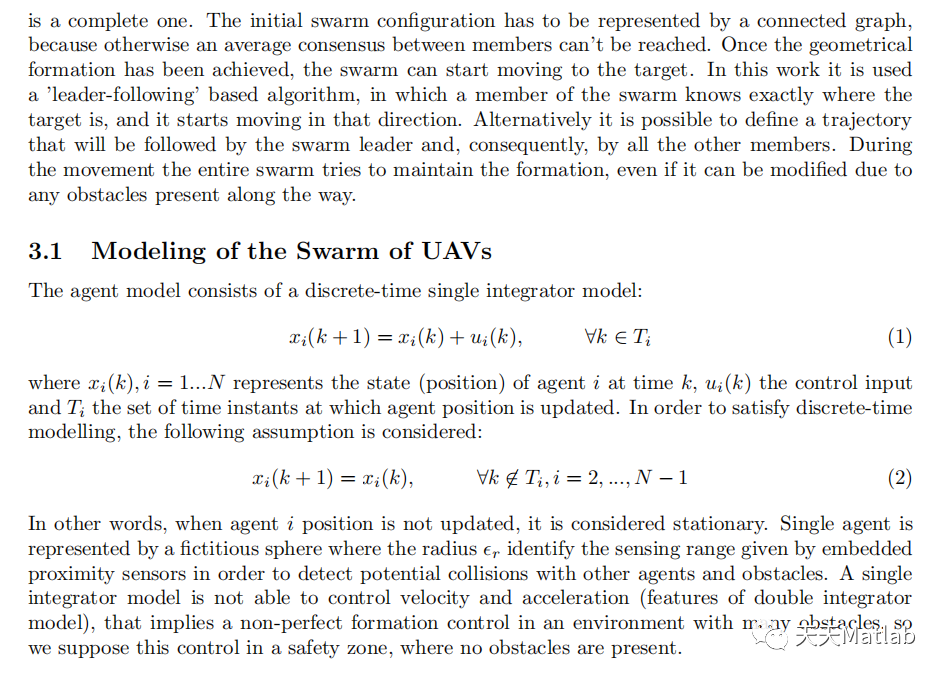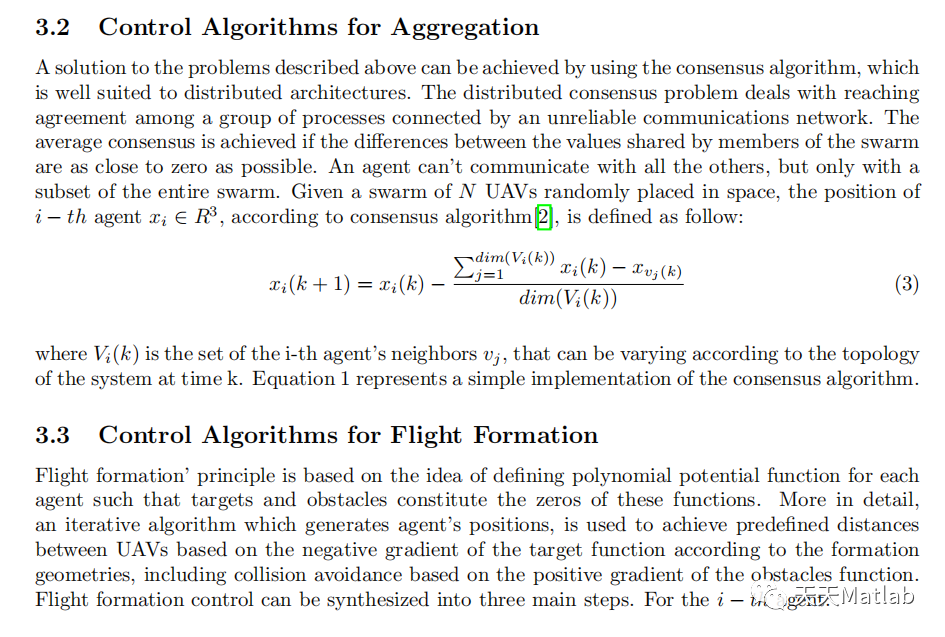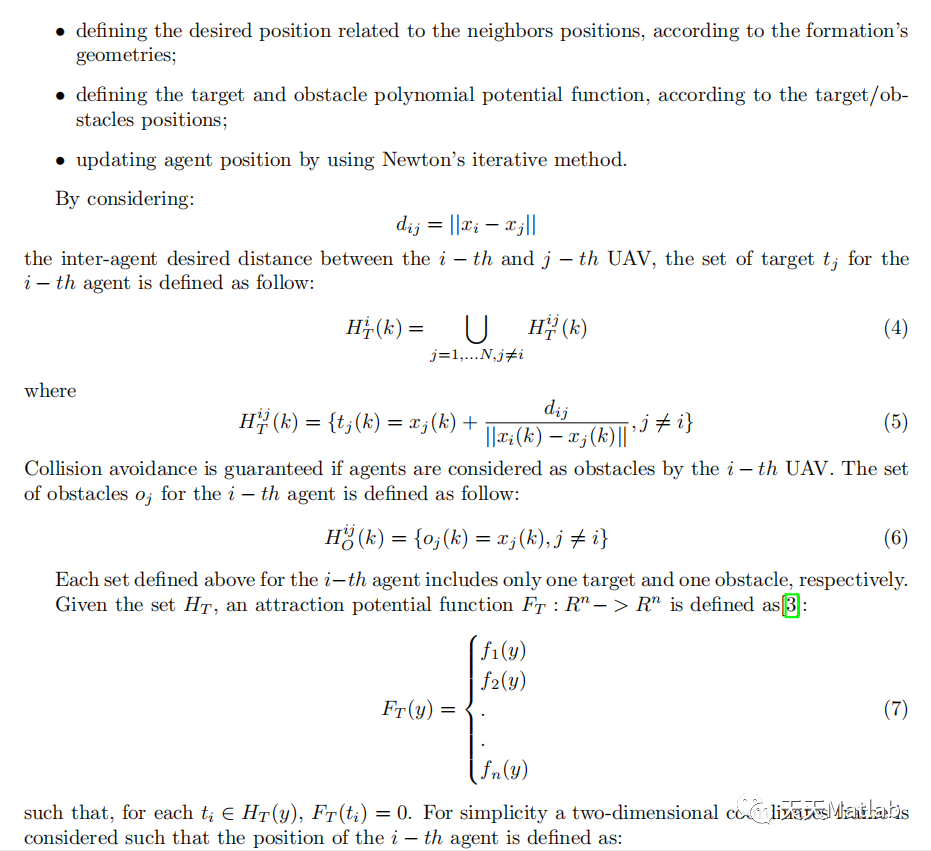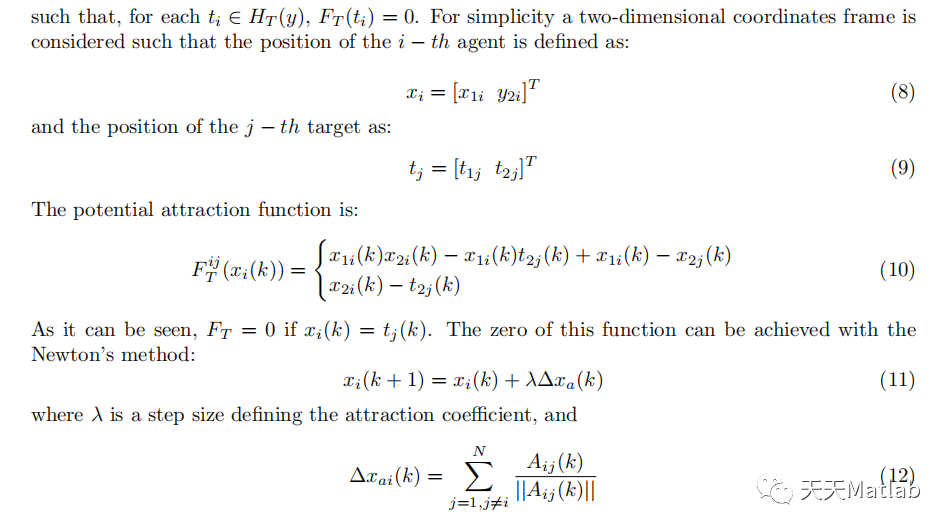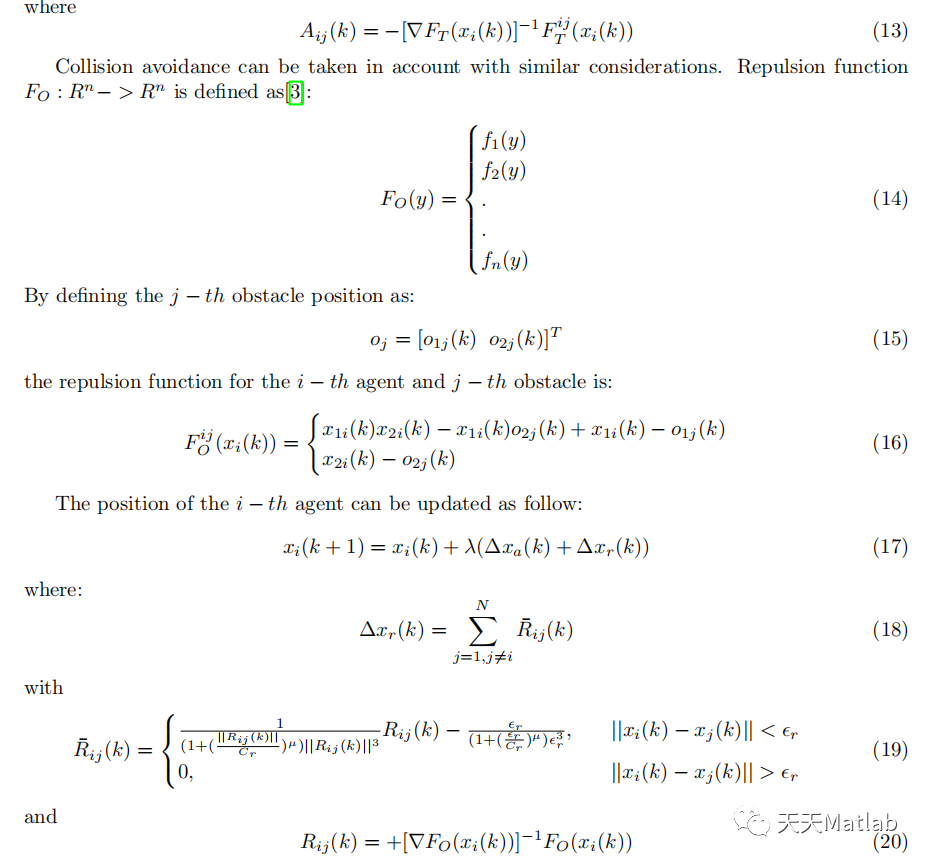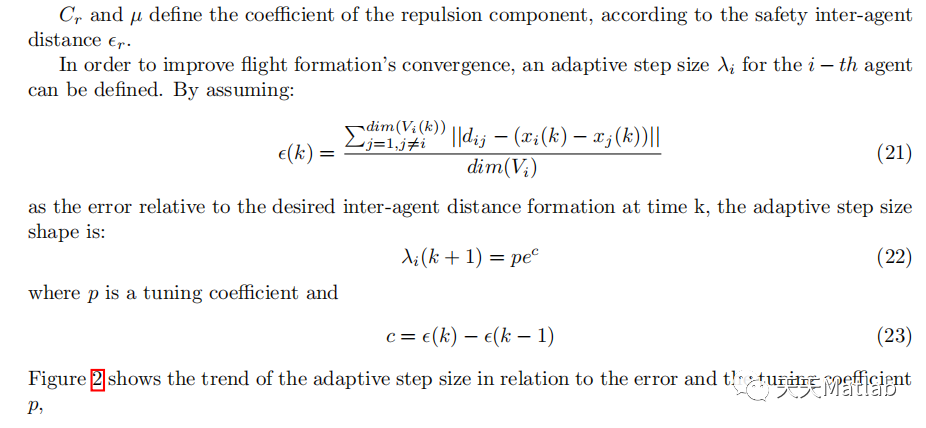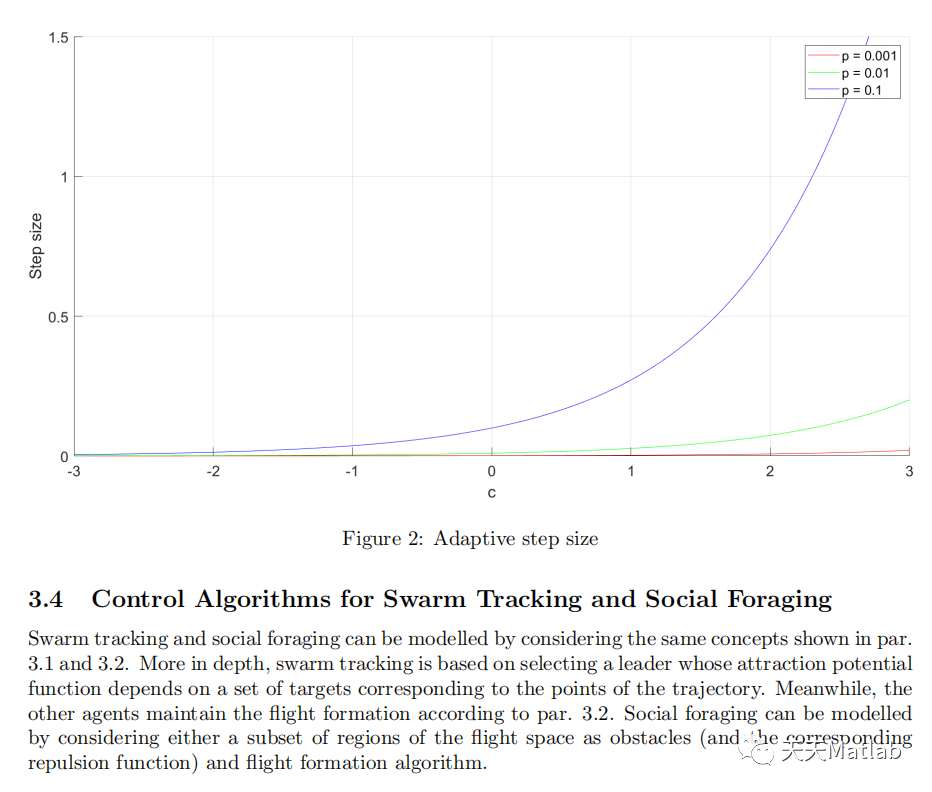2 部分代码
clear all
close all
clc
syms x1
syms x2
grid on
hold on
axis equal

N = 4;
d = 1.5;
pos = 3*rand(2,N);
pos = [pos; rand(1,N)];
pos(1:2,1) = [5;0];
[pos,obst,target,dist_matrix,lambda,sec_dist,mu,Cr,d,tr1,tr2,tr3,tr4] = formazione_rombo(pos,d,[],[],1,0.02);
initial_pos = pos;
plot3(initial_pos(1,1),initial_pos(2,1),initial_pos(3,1),'s','MarkerSize',10, 'MarkerFaceColor','y','HandleVisibility','off');
plot3(initial_pos(1,2:4),initial_pos(2,2:4),initial_pos(3,2:4),'s','MarkerSize',10, 'MarkerFaceColor','g');
G = create_graph(pos,sqrt(2)*d);
while norm(pos(:,1)-target(:,1)) > 0.1
G = create_graph(pos,sqrt(2)*d);
check = checkDist(pos,dist_matrix,G)

%ripristino la formazione se si sminchia troppo
while check > 2
G = create_graph(pos,sqrt(2)*d);
check = checkDist(pos,dist_matrix,G)
end

tr1 = [[tr1] [pos(:,1)]];
tr2 = [[tr2] [pos(:,2)]];
tr3 = [[tr3] [pos(:,3)]];
tr4 = [[tr4] [pos(:,4)]];
disp(norm(pos(:,1)-target(:,1)));
end
G = create_graph(pos,sqrt(2)*d);
check = checkDist(pos,dist_matrix,G);
if check >0.15
[pos,~,~,~,~,~,~,~,~,tr11,tr22,tr33,tr44] = formazione_rombo(pos,d,[],obst,2,0.02);
else
tr11 = [];
tr22 = [];
tr33 = [];
tr44 = [];
end
tr1 = [tr1 tr11];
tr2 = [tr2 tr22];
tr3 = [tr3 tr33];
tr4 = [tr4 tr44];
plot3(tr1(1,:),tr1(2,:),tr1(3,:),'--');
plot3(pos(1,1),pos(2,1),pos(3,1),'s','MarkerSize',10, 'MarkerFaceColor','y');
plot3(pos(1,2:4),pos(2,2:4),pos(3,2:4),'s','MarkerSize',10, 'MarkerFaceColor','r');
plot3(obst(1,:),obst(2,:),obst(3,:),'*b')
plot3(target(1,1),target(2,1),target(3,1),'-o','MarkerSize',15);
plot3(tr2(1,:),tr2(2,:),tr2(3,:),'--');
plot3(tr3(1,:),tr3(2,:),tr3(3,:),'--');
plot3(tr4(1,:),tr4(2,:),tr4(3,:),'--');


3 仿真结果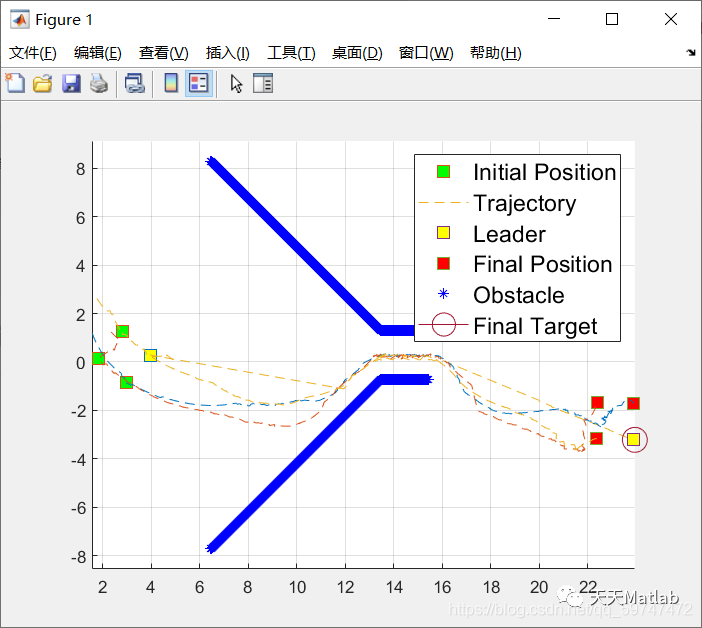展开全文算法
• 1 简介 萤火虫（Glowworms）是一群能发光的昆虫，也被称为闪电虫，它们使用一种叫做生物发光（bioluminescence）的过程来发光。然而，已经发现了许多具有类似发光行为的生物，如水母、某些细菌、原生动物、水生动物...
• 采用蚁群算法的无人机路径规划程序，采用matlab编程。
• 路径规划】基于狼群算法求解无人机三维路径规划matlab源码.md
• 路径问题而言，在具体实施任务时仅靠操作员手中的遥控器控制无人飞行器执行相应的工作，可能会对操作员心理以及技术提出极高的要求，为了避免个人操作失误，进而造成飞行器损坏的危险，一种解决问题的方法就是对...算法
• 为实现无人机编队在指定空域完成从起始点到目标点间的自主路径规划,且机群在该过程中具有良好的协同性和执行高效性,提出了一种无人机群航路自主规划的方法并进行仿真.分析了机群个体的规划路径点及其特点,并通过建立...人工智能
• 使用蚁群算法进行智能避障，并且不断比较出路程，然后寻找出最短路径。此代码有所优化。使用蚁群算法进行智能避障，并且不断比较出路程，然后寻找出最短路径。此代码有所优化。
• 路径规划】基于A星算法的无人机三维路径规划matlab源码
• 1 简介 2 部分代码 closeall; clearall; clc; addpath(genpath('./')); %% Plan path ...%start = { [2 10 2],[1 -4 1],[9.2 17 3],[9.2 10 3],[0.1 10 2]};...% stop = {[1 -4 1],[0.1 17 3],[9 -4...算法
• 多机器人路径规划，避障处理，代码可执行，Matlab软件编制，已经发布prj,可直接添加到MATLAB，作为晓得执行文件执行。
• 无人机路径规划】基于人工势场实现无人机编队路径规划matlab源码.md
• 路径规划】基于BBO算法的无人机三维路径规划matlab源码.zip简介
• 路径规划】基于麻雀搜索算法的无人机三维路径规划matlab 源码.md
• 如专门用作植保的大疆PS-X625无人机，用作街景拍摄与监控巡察的宝鸡行翼航空科技的X8无人机，以及用作水下救援的白鲨MIX水下无人机等，决定飞行器性能主要是内部的飞控系统和外部的路径规划问题。就路径问题而言，在...算法...

# 无人机路径规划matlabmatlab 订阅